Скачать презентацию LECTURE 10 REDOX AND ELECTROCHEMISTRY Figure 4

d4ce56e8bcb874ac829316b77fe5c7a1.ppt

• Количество слайдов: 87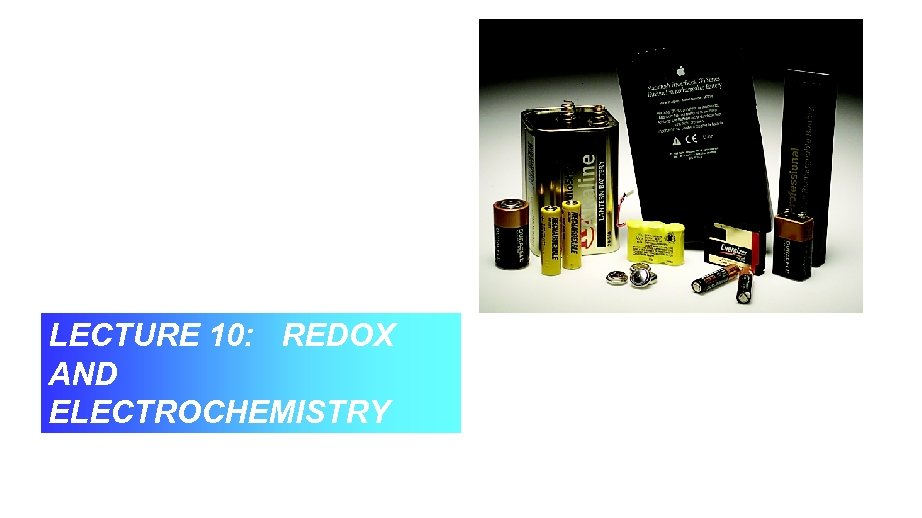LECTURE 10: REDOX AND ELECTROCHEMISTRY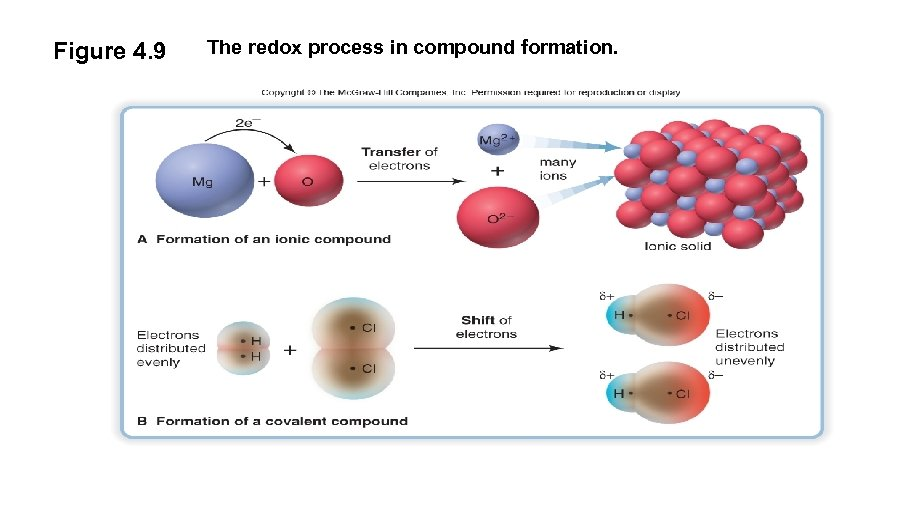Figure 4. 9 The redox process in compound formation.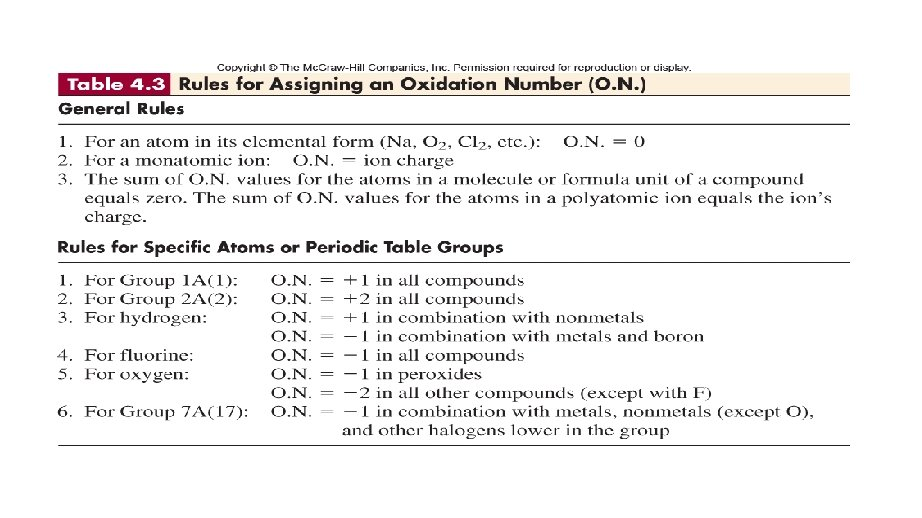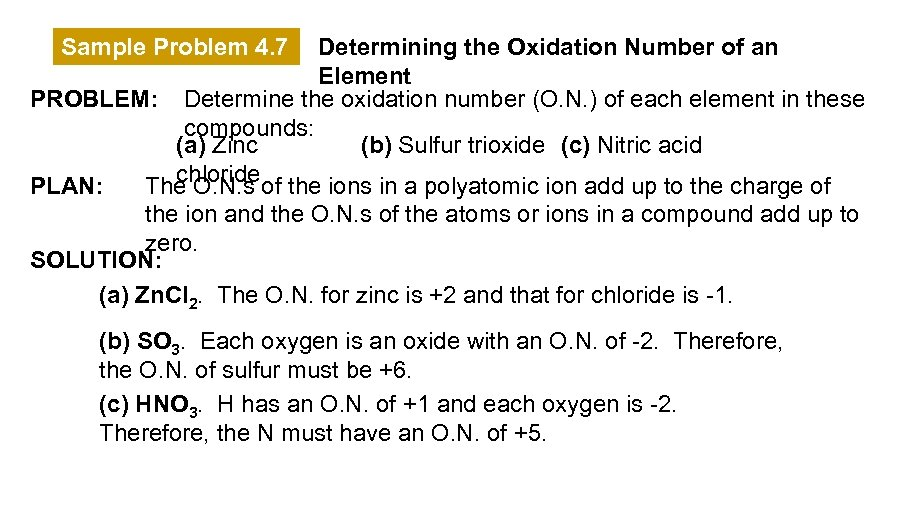Sample Problem 4. 7 Determining the Oxidation Number of an Element PROBLEM: Determine the oxidation number (O. N. ) of each element in these compounds: (a) Zinc (b) Sulfur trioxide (c) Nitric acid chloride PLAN: The O. N. s of the ions in a polyatomic ion add up to the charge of the ion and the O. N. s of the atoms or ions in a compound add up to zero. SOLUTION: (a) Zn. Cl 2. The O. N. for zinc is +2 and that for chloride is -1. (b) SO 3. Each oxygen is an oxide with an O. N. of -2. Therefore, the O. N. of sulfur must be +6. (c) HNO 3. H has an O. N. of +1 and each oxygen is -2. Therefore, the N must have an O. N. of +5.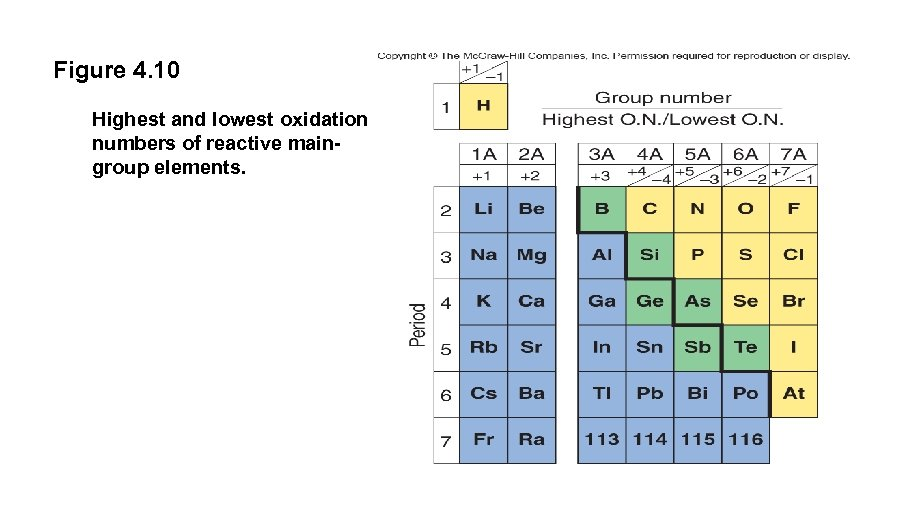Figure 4. 10 Highest and lowest oxidation numbers of reactive maingroup elements.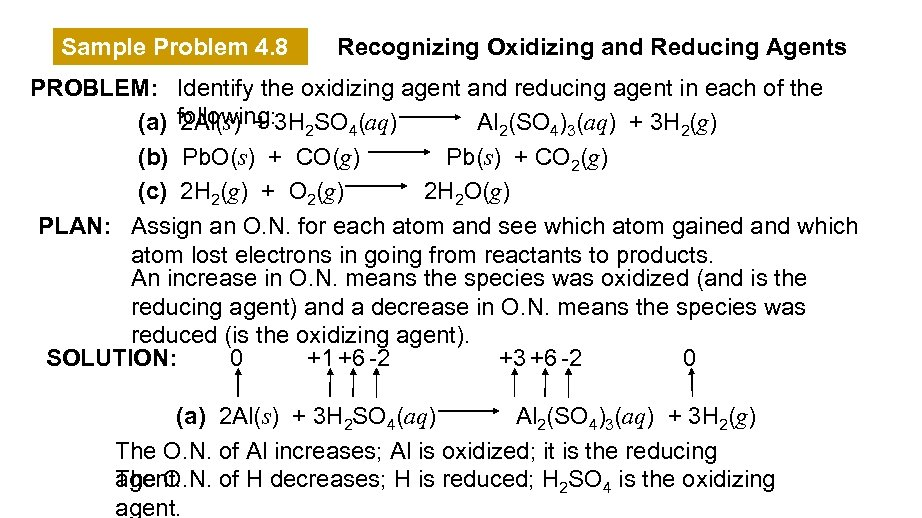Sample Problem 4. 8 Recognizing Oxidizing and Reducing Agents PROBLEM: Identify the oxidizing agent and reducing agent in each of the (a) following: 3 H 2 SO 4(aq) 2 Al(s) + Al 2(SO 4)3(aq) + 3 H 2(g) (b) Pb. O(s) + CO(g) Pb(s) + CO 2(g) (c) 2 H 2(g) + O 2(g) 2 H 2 O(g) PLAN: Assign an O. N. for each atom and see which atom gained and which atom lost electrons in going from reactants to products. An increase in O. N. means the species was oxidized (and is the reducing agent) and a decrease in O. N. means the species was reduced (is the oxidizing agent). SOLUTION: 0 +1 +6 -2 +3 +6 -2 0 (a) 2 Al(s) + 3 H 2 SO 4(aq) Al 2(SO 4)3(aq) + 3 H 2(g) The O. N. of Al increases; Al is oxidized; it is the reducing The O. N. of H decreases; H is reduced; H 2 SO 4 is the oxidizing agent.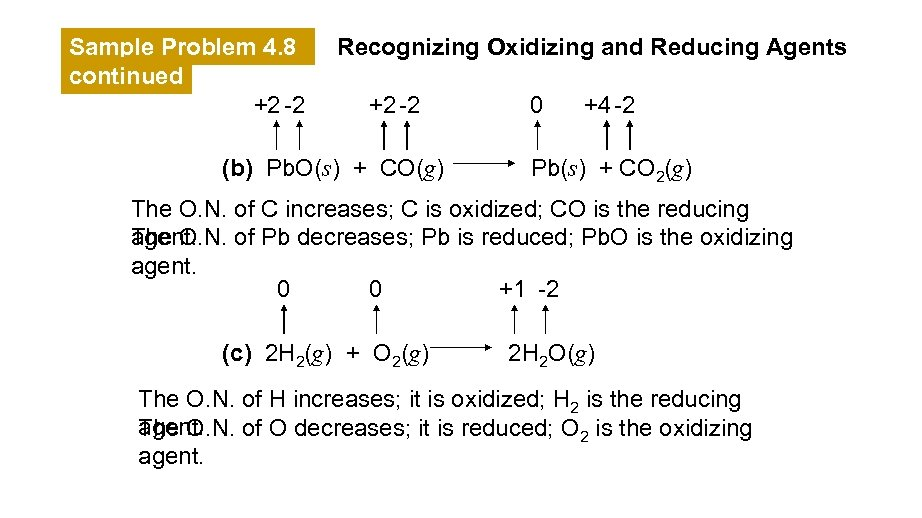Sample Problem 4. 8 continued +2 -2 Recognizing Oxidizing and Reducing Agents +2 -2 (b) Pb. O(s) + CO(g) 0 +4 -2 Pb(s) + CO 2(g) The O. N. of C increases; C is oxidized; CO is the reducing The O. N. of Pb decreases; Pb is reduced; Pb. O is the oxidizing agent. 0 0 +1 -2 (c) 2 H 2(g) + O 2(g) 2 H 2 O(g) The O. N. of H increases; it is oxidized; H 2 is the reducing agent. The O. N. of O decreases; it is reduced; O 2 is the oxidizing agent.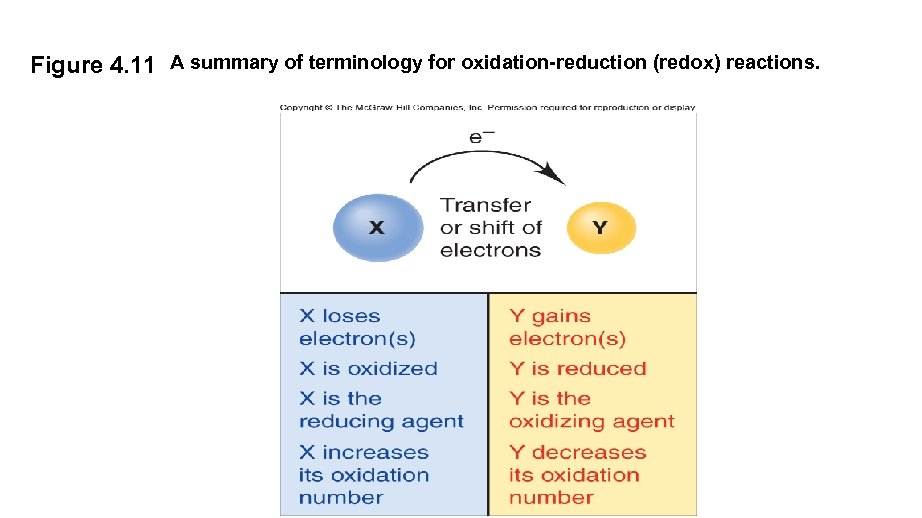Figure 4. 11 A summary of terminology for oxidation-reduction (redox) reactions.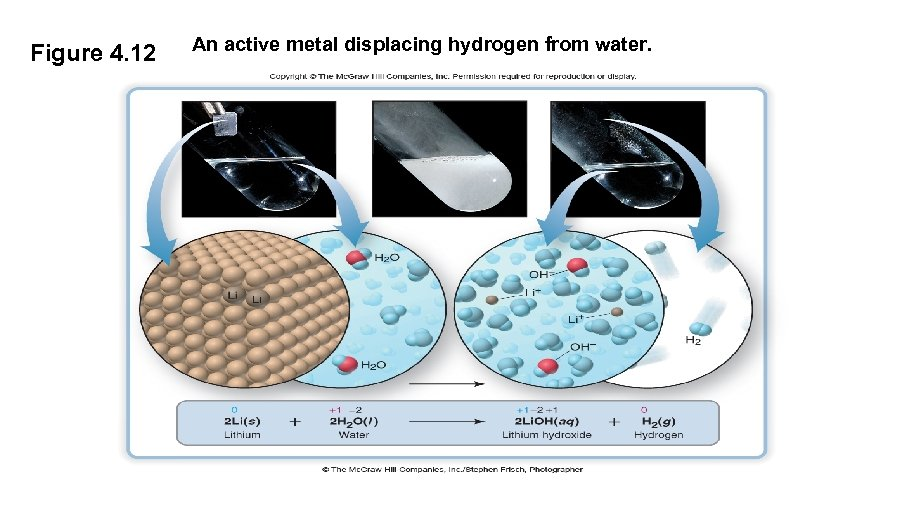Figure 4. 12 An active metal displacing hydrogen from water.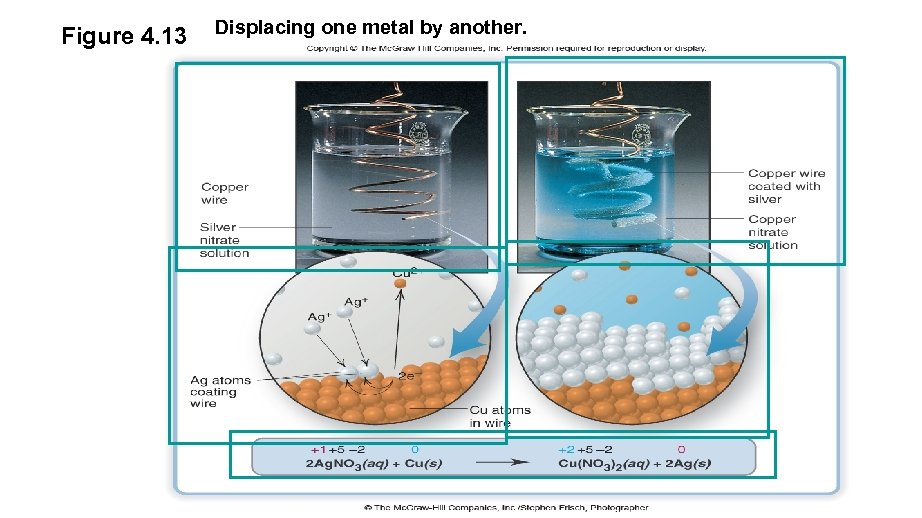Figure 4. 13 Displacing one metal by another.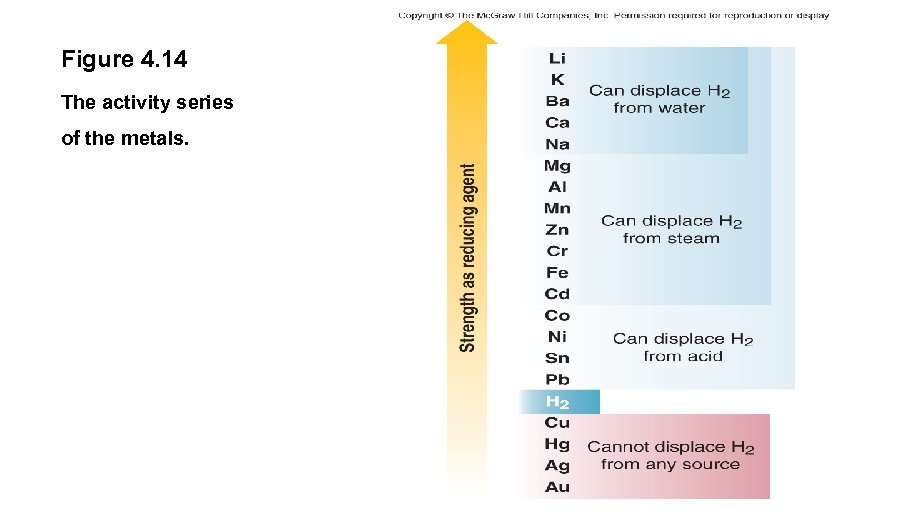Figure 4. 14 The activity series of the metals.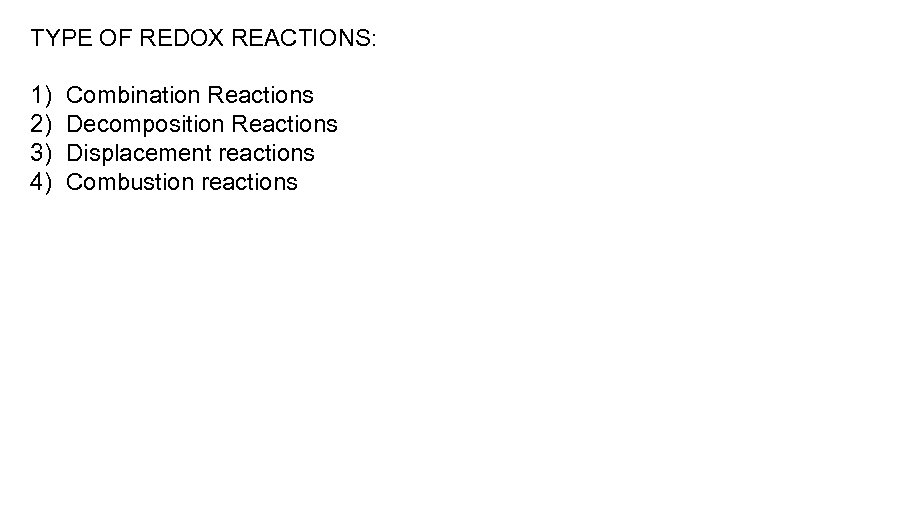TYPE OF REDOX REACTIONS: 1) 2) 3) 4) Combination Reactions Decomposition Reactions Displacement reactions Combustion reactions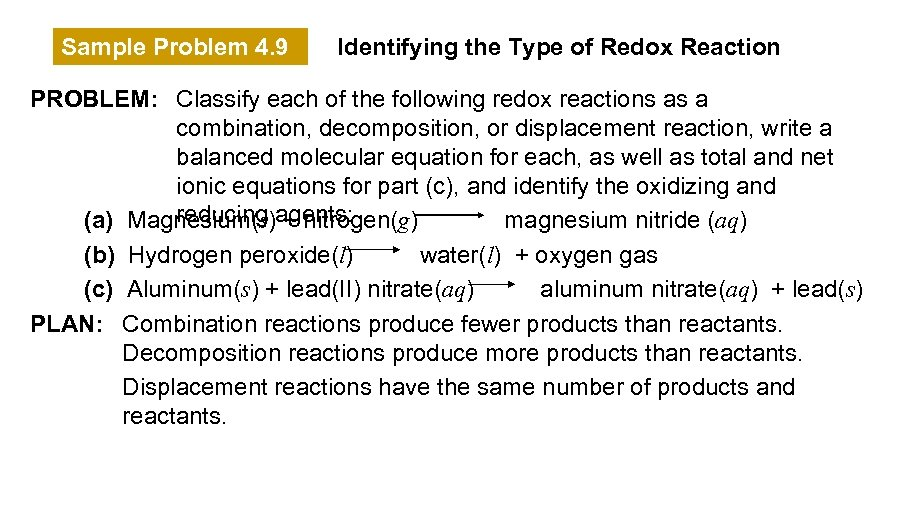Sample Problem 4. 9 Identifying the Type of Redox Reaction PROBLEM: Classify each of the following redox reactions as a combination, decomposition, or displacement reaction, write a balanced molecular equation for each, as well as total and net ionic equations for part (c), and identify the oxidizing and reducing + nitrogen(g) (a) Magnesium(s)agents: magnesium nitride (aq) (b) Hydrogen peroxide(l) water(l) + oxygen gas (c) Aluminum(s) + lead(II) nitrate(aq) aluminum nitrate(aq) + lead(s) PLAN: Combination reactions produce fewer products than reactants. Decomposition reactions produce more products than reactants. Displacement reactions have the same number of products and reactants.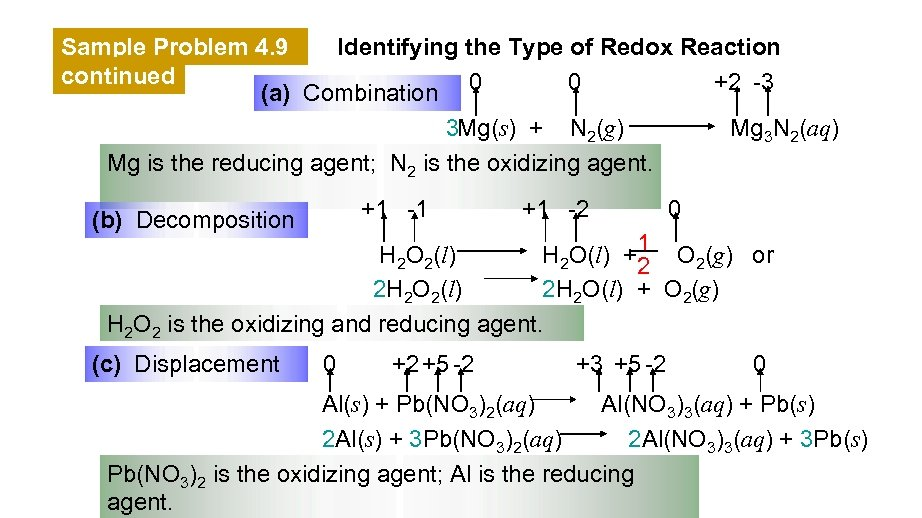Sample Problem 4. 9 Identifying the Type of Redox Reaction continued 0 +2 -3 (a) Combination 0 Mg 3 N 2(aq) 3 Mg(s) + N 2(g) Mg is the reducing agent; N 2 is the oxidizing agent. +1 -1 (b) Decomposition +1 -2 0 H 2 O 2(l) H 2 O(l) +1 O 2(g) or 2 2 H 2 O 2(l) 2 H 2 O(l) + O 2(g) H 2 O 2 is the oxidizing and reducing agent. (c) Displacement 0 +2 +5 -2 +3 +5 -2 0 Al(s) + Pb(NO 3)2(aq) Al(NO 3)3(aq) + Pb(s) 2 Al(s) + 3 Pb(NO 3)2(aq) 2 Al(NO 3)3(aq) + 3 Pb(s) Pb(NO 3)2 is the oxidizing agent; Al is the reducing agent.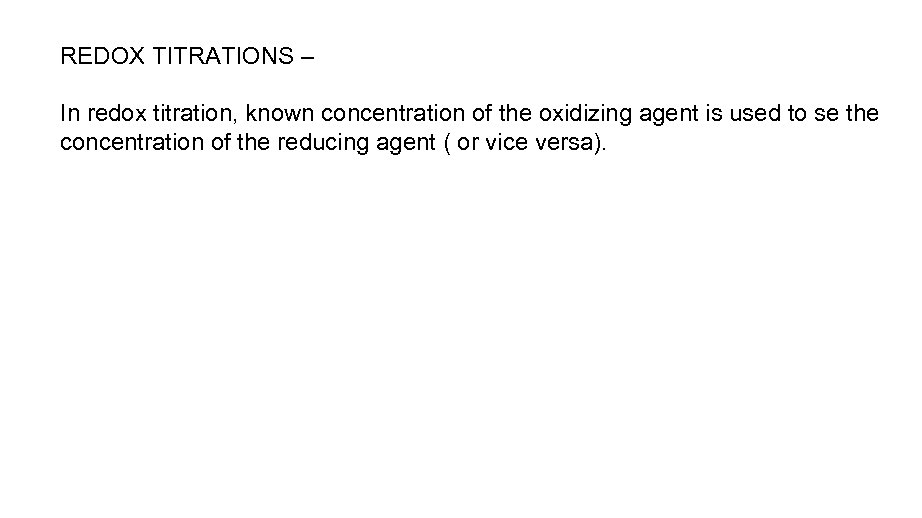REDOX TITRATIONS – In redox titration, known concentration of the oxidizing agent is used to se the concentration of the reducing agent ( or vice versa).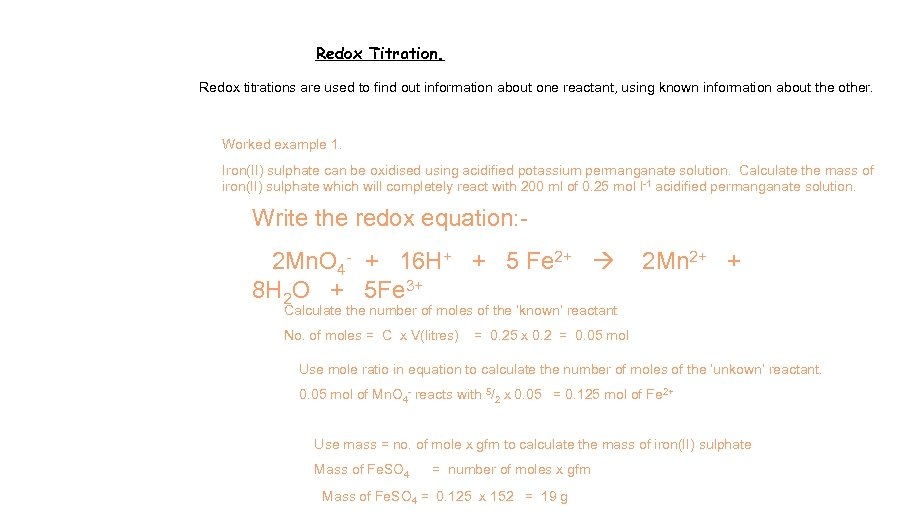Redox Titration. Redox titrations are used to find out information about one reactant, using known information about the other. Worked example 1. Iron(II) sulphate can be oxidised using acidified potassium permanganate solution. Calculate the mass of iron(II) sulphate which will completely react with 200 ml of 0. 25 mol l-1 acidified permanganate solution. Write the redox equation: 2 Mn. O 4 - + 16 H+ + 5 Fe 2+ 8 H 2 O + 5 Fe 3+ 2 Mn 2+ + Calculate the number of moles of the ‘known’ reactant No. of moles = C x V(litres) = 0. 25 x 0. 2 = 0. 05 mol Use mole ratio in equation to calculate the number of moles of the ‘unkown’ reactant. 0. 05 mol of Mn. O 4 - reacts with 5/2 x 0. 05 = 0. 125 mol of Fe 2+ Use mass = no. of mole x gfm to calculate the mass of iron(II) sulphate Mass of Fe. SO 4 = number of moles x gfm Mass of Fe. SO 4 = 0. 125 x 152 = 19 g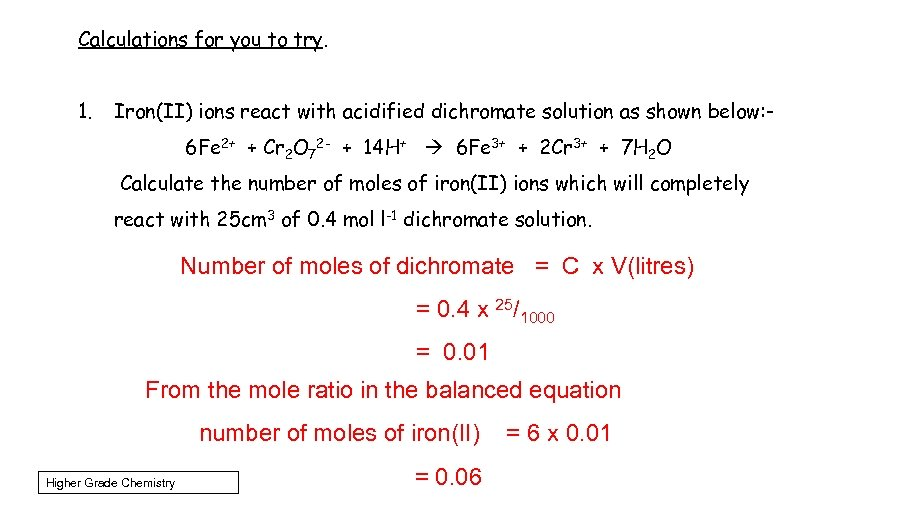Calculations for you to try. 1. Iron(II) ions react with acidified dichromate solution as shown below: 6 Fe 2+ + Cr 2 O 72 - + 14 H+ 6 Fe 3+ + 2 Cr 3+ + 7 H 2 O Calculate the number of moles of iron(II) ions which will completely react with 25 cm 3 of 0. 4 mol l-1 dichromate solution. Number of moles of dichromate = C x V(litres) = 0. 4 x 25/1000 = 0. 01 From the mole ratio in the balanced equation number of moles of iron(II) Higher Grade Chemistry = 0. 06 = 6 x 0. 01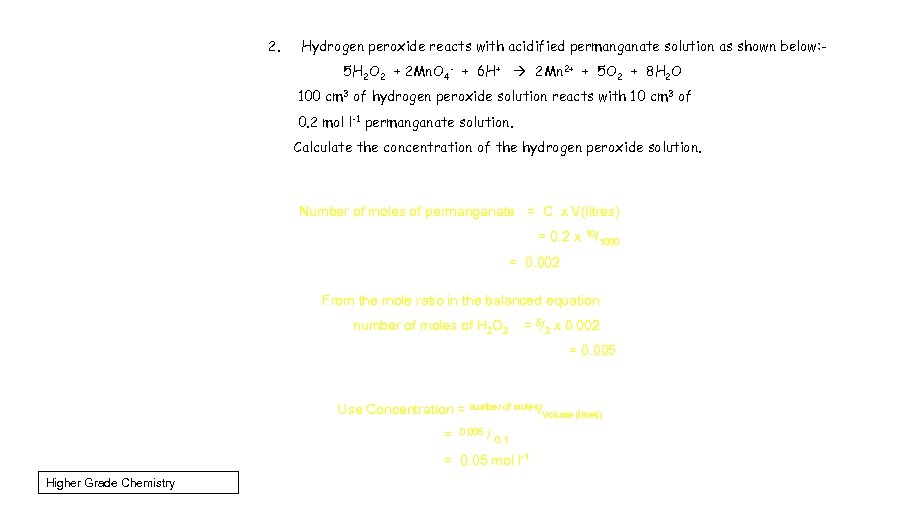2. Hydrogen peroxide reacts with acidified permanganate solution as shown below: 5 H 2 O 2 + 2 Mn. O 4 - + 6 H+ 2 Mn 2+ + 5 O 2 + 8 H 2 O 100 cm 3 of hydrogen peroxide solution reacts with 10 cm 3 of 0. 2 mol l-1 permanganate solution. Calculate the concentration of the hydrogen peroxide solution. Number of moles of permanganate = C x V(litres) = 0. 2 x 10/1000 = 0. 002 From the mole ratio in the balanced equation number of moles of H 2 O 2 = 5/2 x 0. 002 = 0. 005 Use Concentration = number of moles/Volume (litres) = 0. 005 / 0. 1 = 0. 05 mol l-1 Higher Grade Chemistry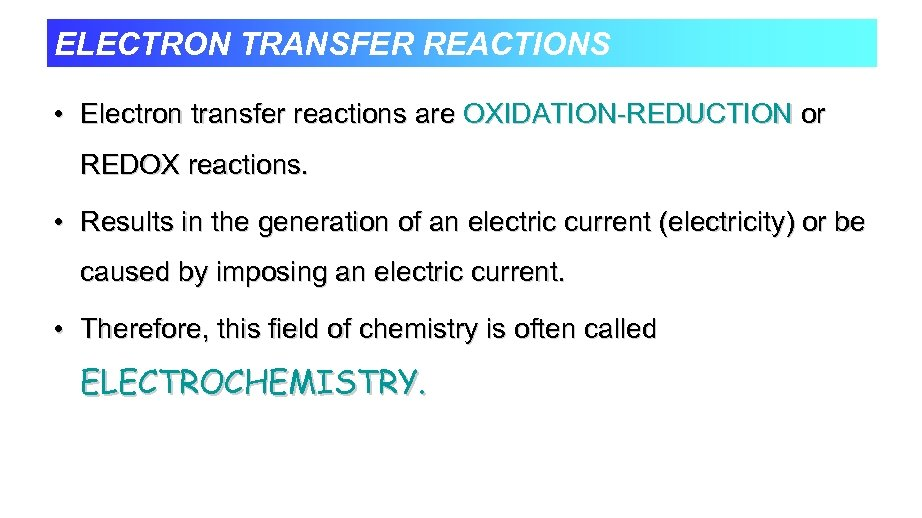ELECTRON TRANSFER REACTIONS • Electron transfer reactions are OXIDATION-REDUCTION or REDOX reactions. • Results in the generation of an electric current (electricity) or be caused by imposing an electric current. • Therefore, this field of chemistry is often called ELECTROCHEMISTRY.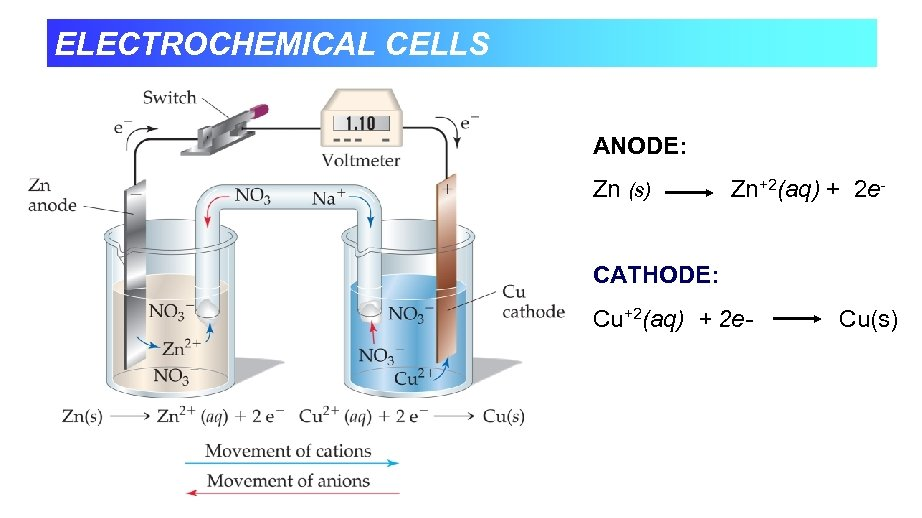ELECTROCHEMICAL CELLS ANODE: Zn (s) Zn+2(aq) + 2 e- CATHODE: Cu+2(aq) + 2 e- Cu(s)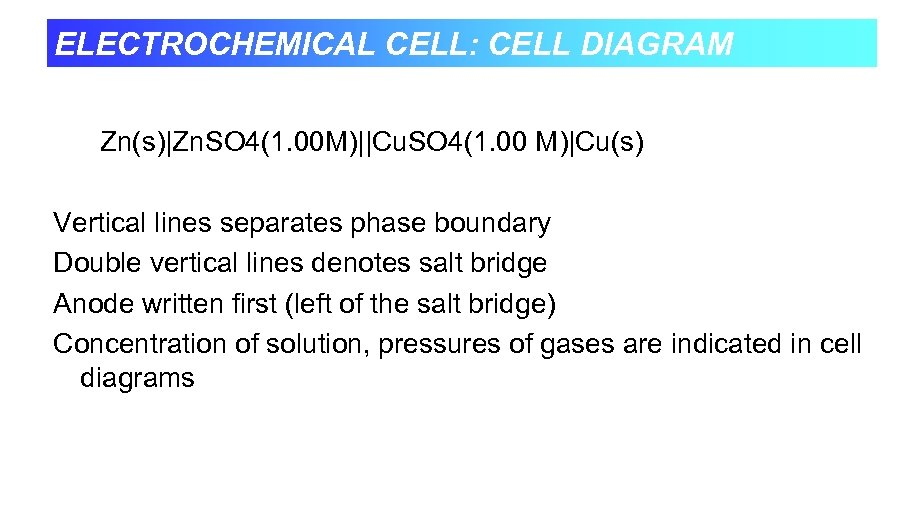ELECTROCHEMICAL CELL: CELL DIAGRAM Zn(s)|Zn. SO 4(1. 00 M)||Cu. SO 4(1. 00 M)|Cu(s) Vertical lines separates phase boundary Double vertical lines denotes salt bridge Anode written first (left of the salt bridge) Concentration of solution, pressures of gases are indicated in cell diagrams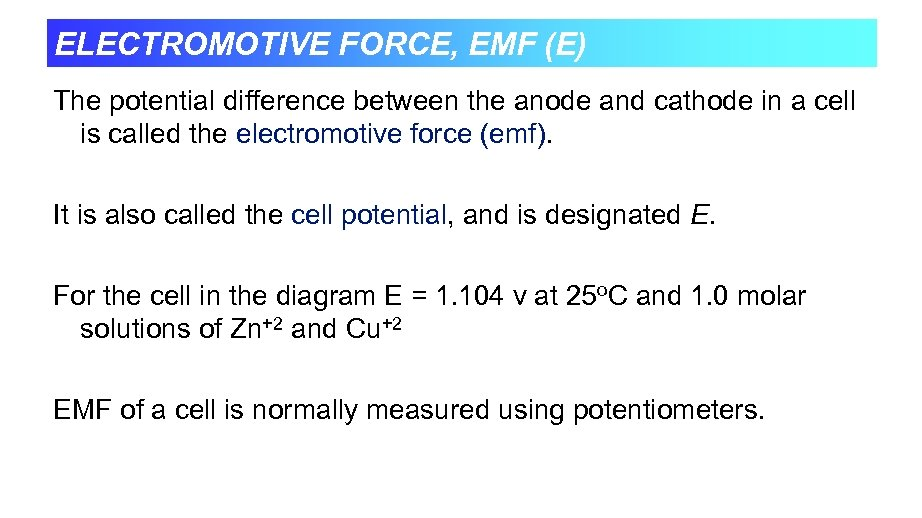ELECTROMOTIVE FORCE, EMF (E) The potential difference between the anode and cathode in a cell is called the electromotive force (emf). It is also called the cell potential, and is designated E. For the cell in the diagram E = 1. 104 v at 25 o. C and 1. 0 molar solutions of Zn+2 and Cu+2 EMF of a cell is normally measured using potentiometers.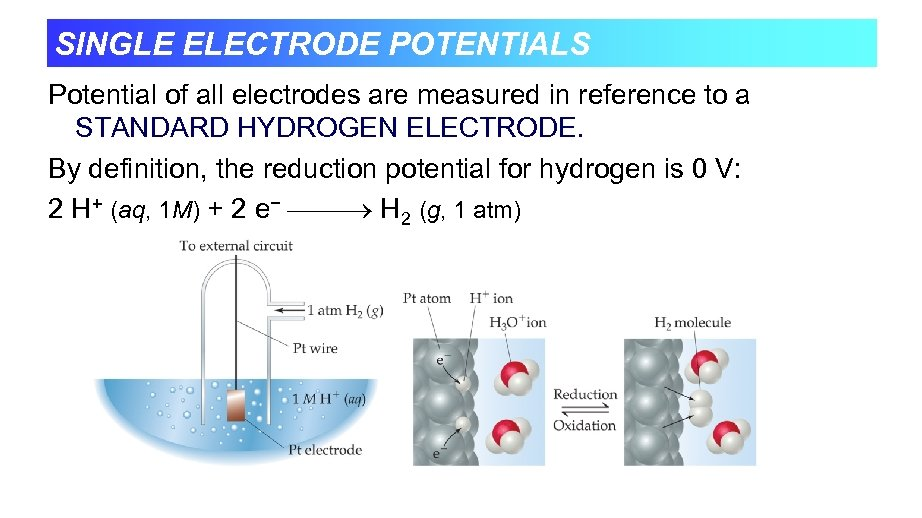SINGLE ELECTRODE POTENTIALS Potential of all electrodes are measured in reference to a STANDARD HYDROGEN ELECTRODE. By definition, the reduction potential for hydrogen is 0 V: 2 H+ (aq, 1 M) + 2 e− H 2 (g, 1 atm)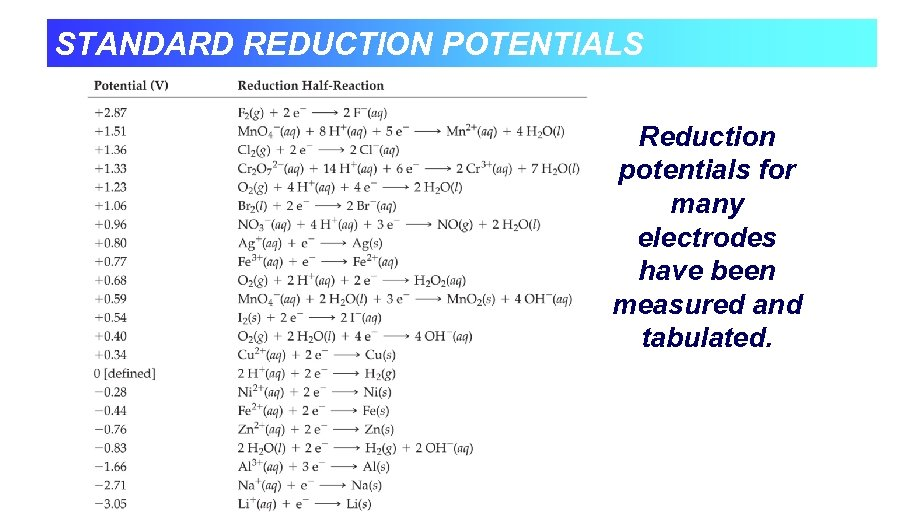STANDARD REDUCTION POTENTIALS Reduction potentials for many electrodes have been measured and tabulated.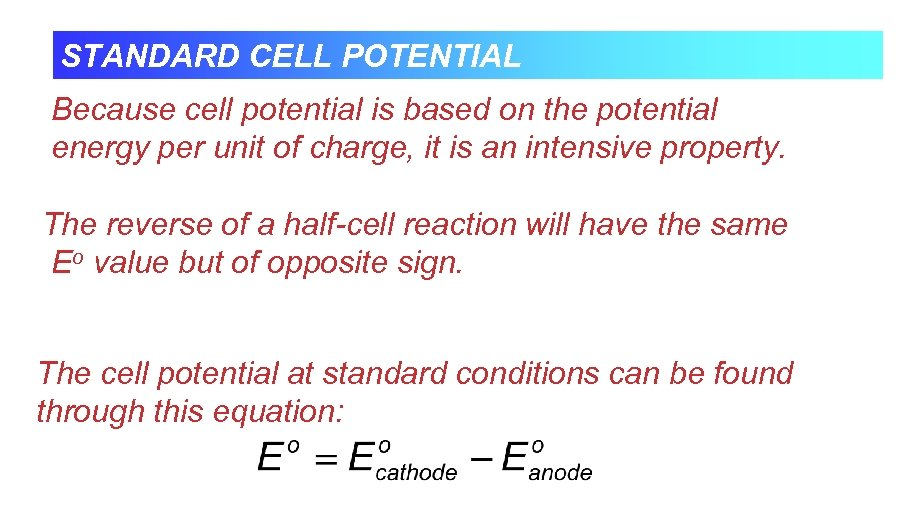STANDARD CELL POTENTIAL Because cell potential is based on the potential energy per unit of charge, it is an intensive property. The reverse of a half-cell reaction will have the same Eo value but of opposite sign. The cell potential at standard conditions can be found through this equation: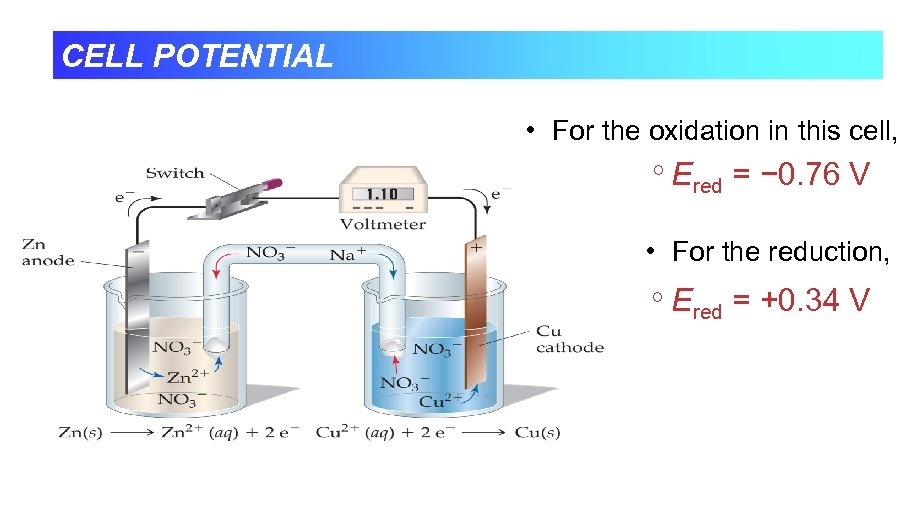CELL POTENTIAL • For the oxidation in this cell, Ered = − 0. 76 V • For the reduction, Ered = +0. 34 V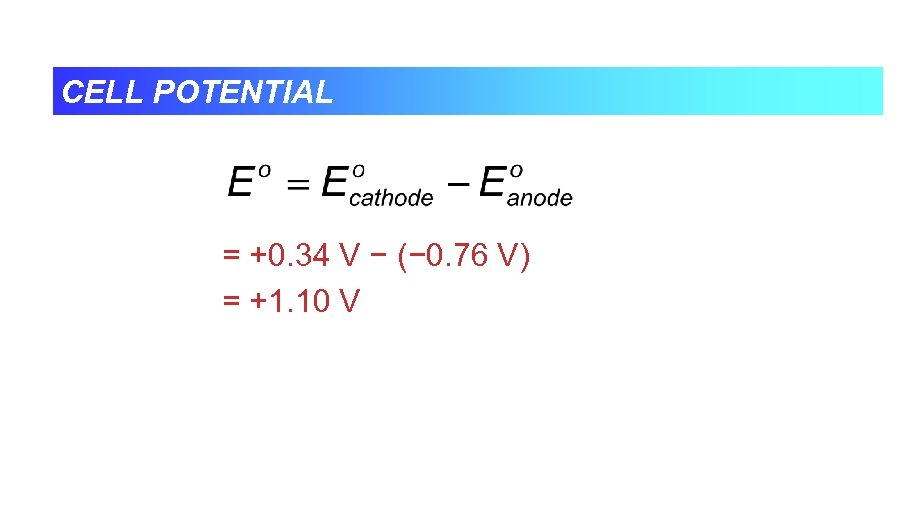CELL POTENTIAL = +0. 34 V − (− 0. 76 V) = +1. 10 V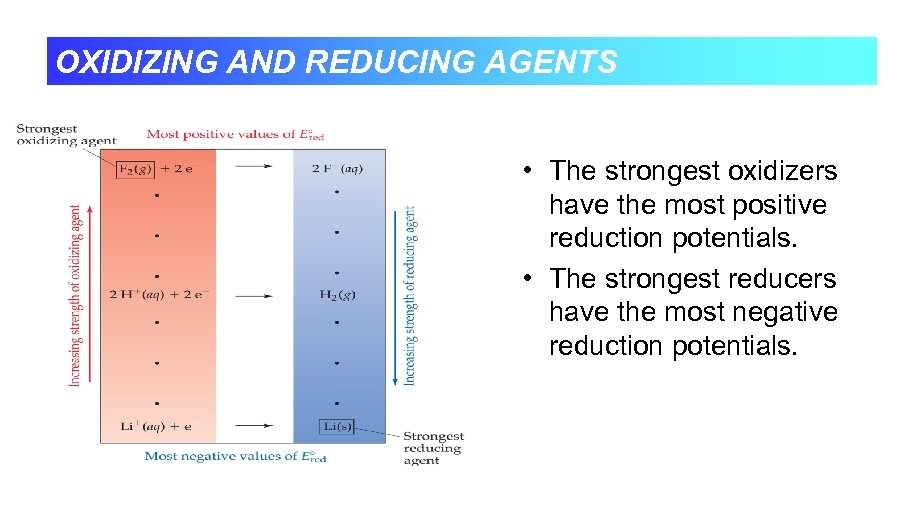OXIDIZING AND REDUCING AGENTS • The strongest oxidizers have the most positive reduction potentials. • The strongest reducers have the most negative reduction potentials.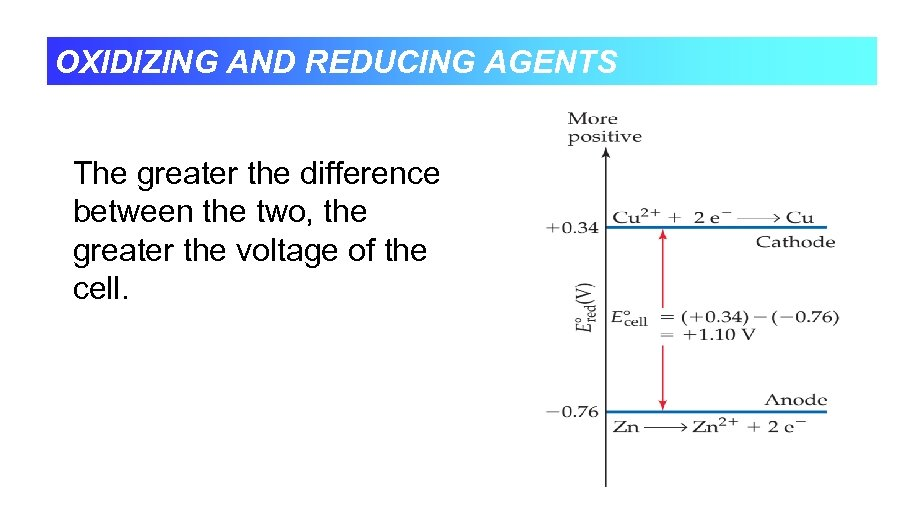OXIDIZING AND REDUCING AGENTS The greater the difference between the two, the greater the voltage of the cell.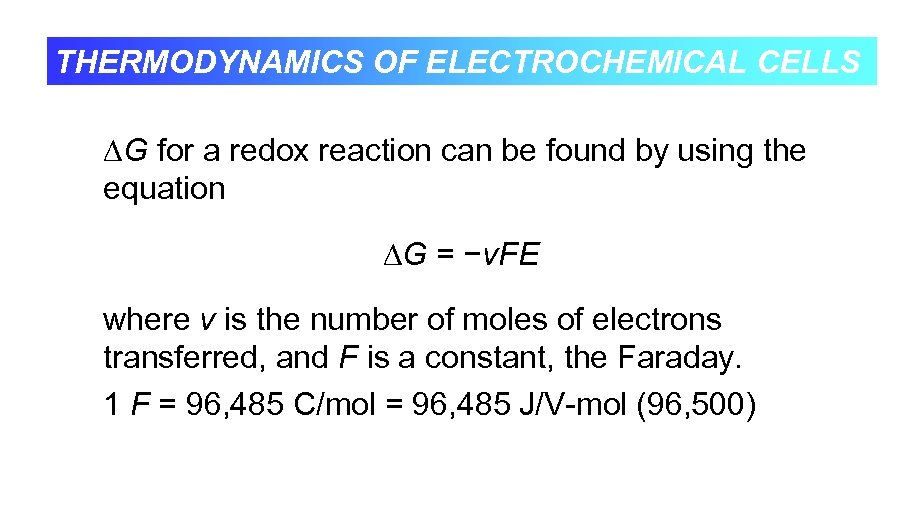THERMODYNAMICS OF ELECTROCHEMICAL CELLS G for a redox reaction can be found by using the equation G = −v. FE where v is the number of moles of electrons transferred, and F is a constant, the Faraday. 1 F = 96, 485 C/mol = 96, 485 J/V-mol (96, 500)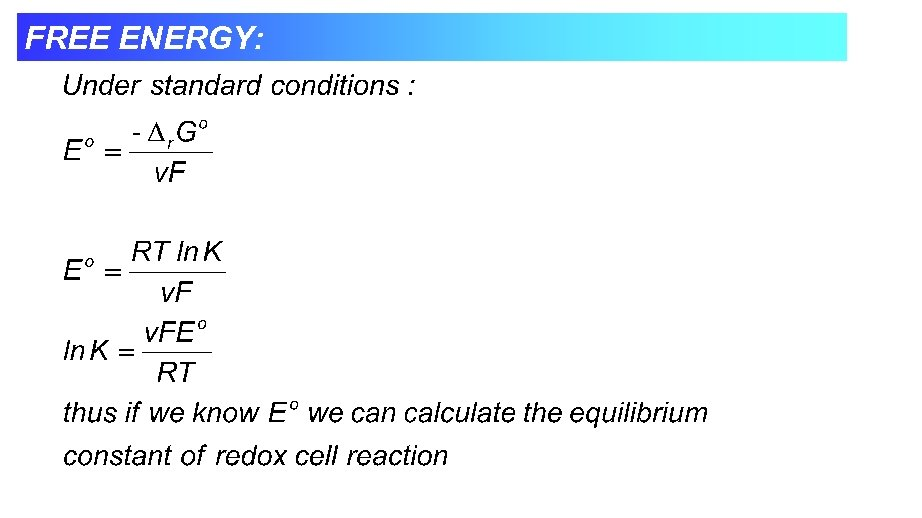FREE ENERGY: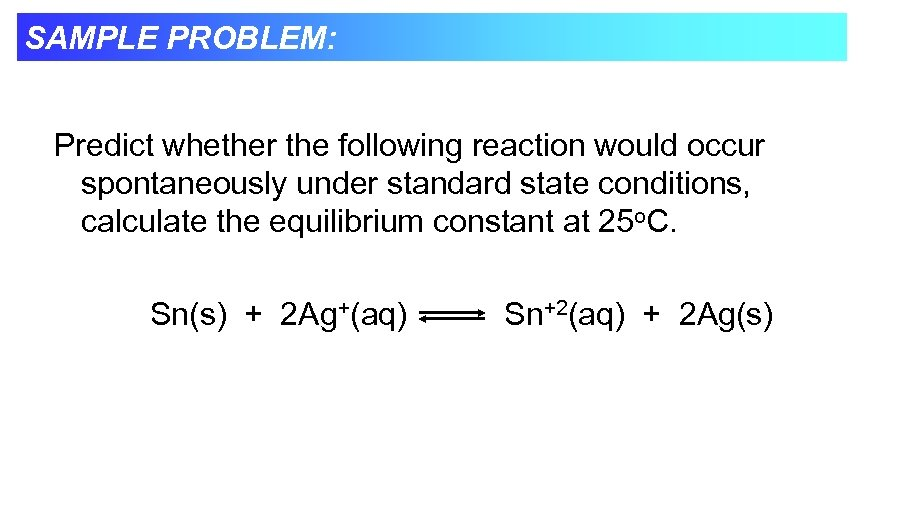SAMPLE PROBLEM: Predict whether the following reaction would occur spontaneously under standard state conditions, calculate the equilibrium constant at 25 o. C. Sn(s) + 2 Ag+(aq) Sn+2(aq) + 2 Ag(s)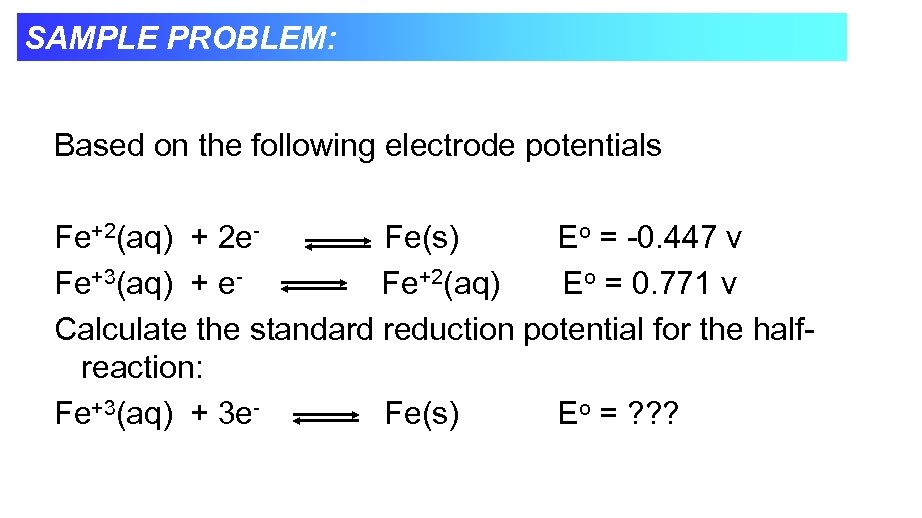SAMPLE PROBLEM: Based on the following electrode potentials Fe+2(aq) + 2 e. Fe(s) Eo = -0. 447 v Fe+3(aq) + e. Fe+2(aq) Eo = 0. 771 v Calculate the standard reduction potential for the halfreaction: Fe+3(aq) + 3 e. Fe(s) Eo = ? ? ?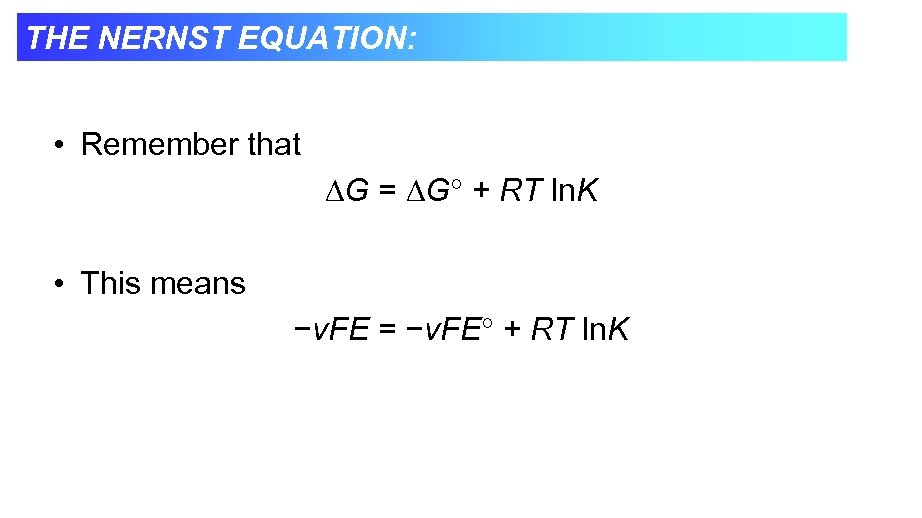THE NERNST EQUATION: • Remember that G = G + RT ln. K • This means −v. FE = −v. FE + RT ln. K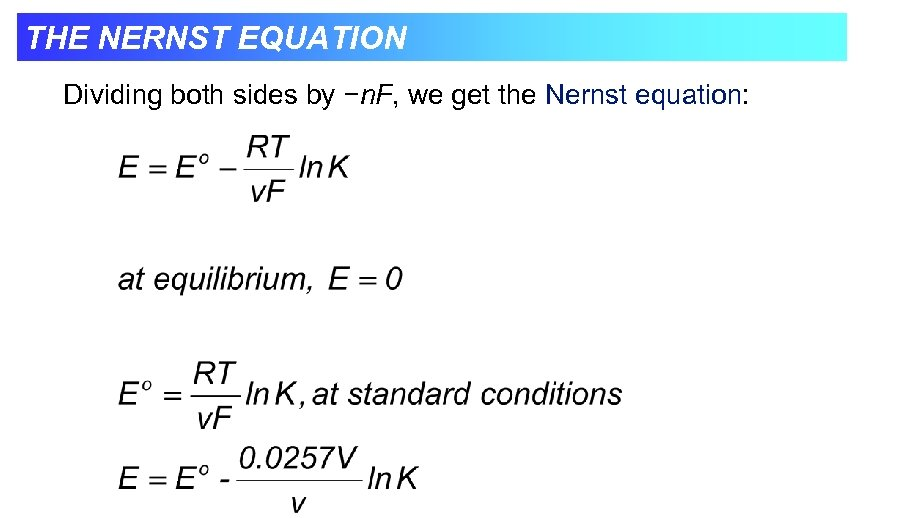THE NERNST EQUATION Dividing both sides by −n. F, we get the Nernst equation: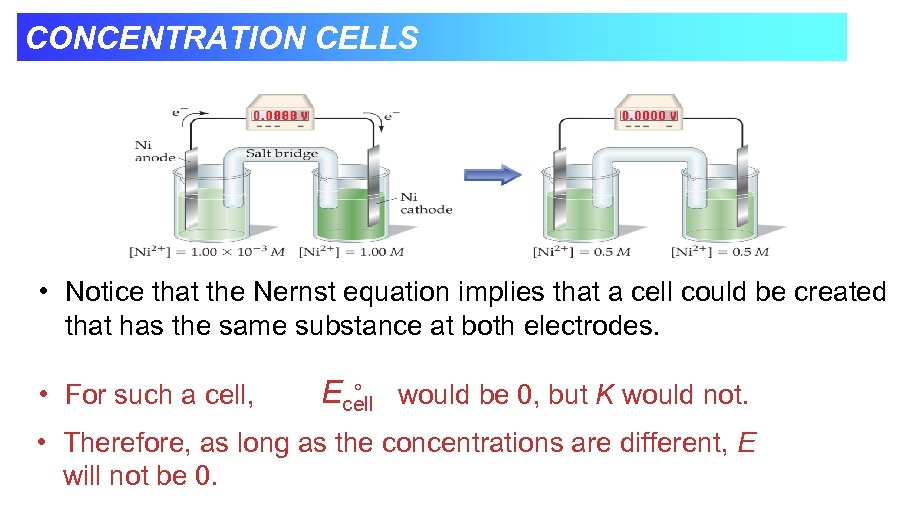CONCENTRATION CELLS • Notice that the Nernst equation implies that a cell could be created that has the same substance at both electrodes. • For such a cell, Ecell would be 0, but K would not. • Therefore, as long as the concentrations are different, E will not be 0.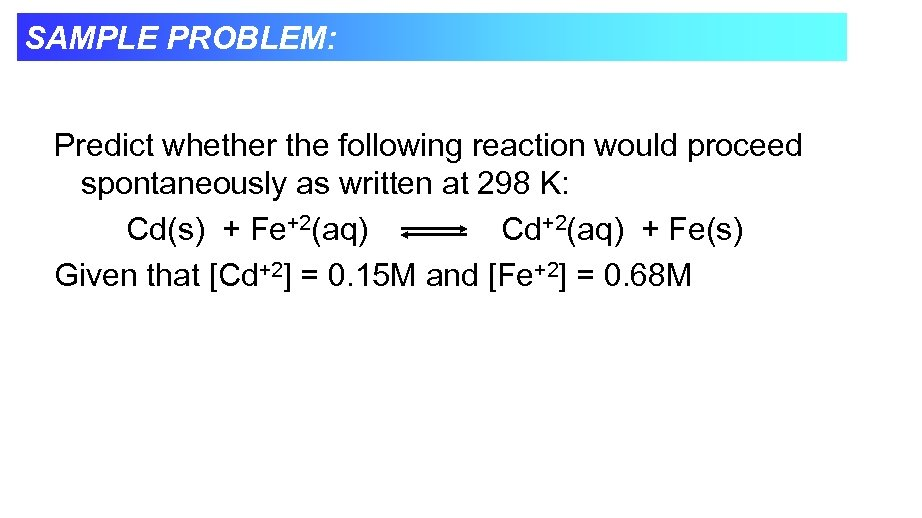SAMPLE PROBLEM: Predict whether the following reaction would proceed spontaneously as written at 298 K: Cd(s) + Fe+2(aq) Cd+2(aq) + Fe(s) Given that [Cd+2] = 0. 15 M and [Fe+2] = 0. 68 M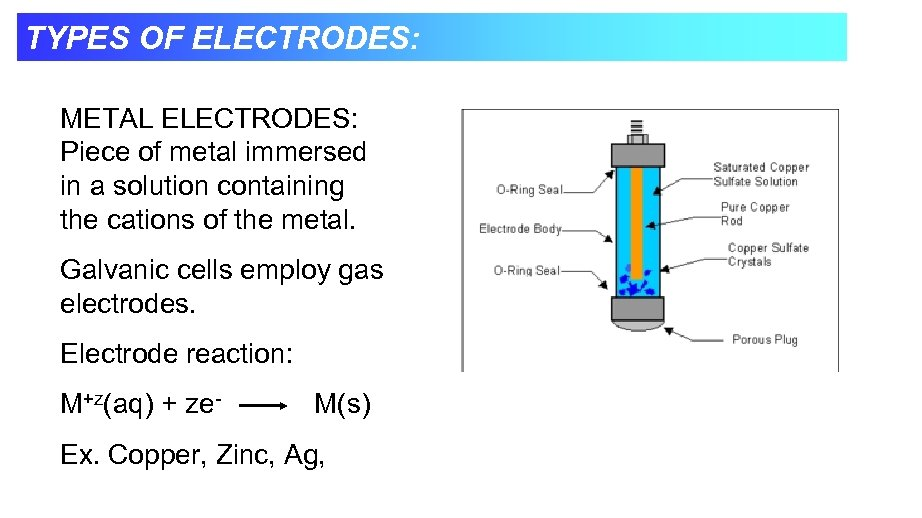TYPES OF ELECTRODES: METAL ELECTRODES: Piece of metal immersed in a solution containing the cations of the metal. Galvanic cells employ gas electrodes. Electrode reaction: M+z(aq) + ze- M(s) Ex. Copper, Zinc, Ag,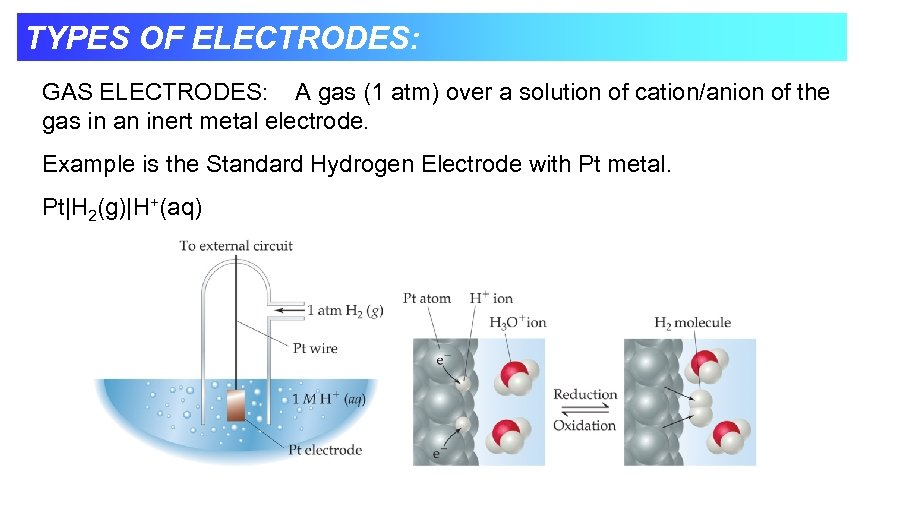TYPES OF ELECTRODES: GAS ELECTRODES: A gas (1 atm) over a solution of cation/anion of the gas in an inert metal electrode. Example is the Standard Hydrogen Electrode with Pt metal. Pt|H 2(g)|H+(aq)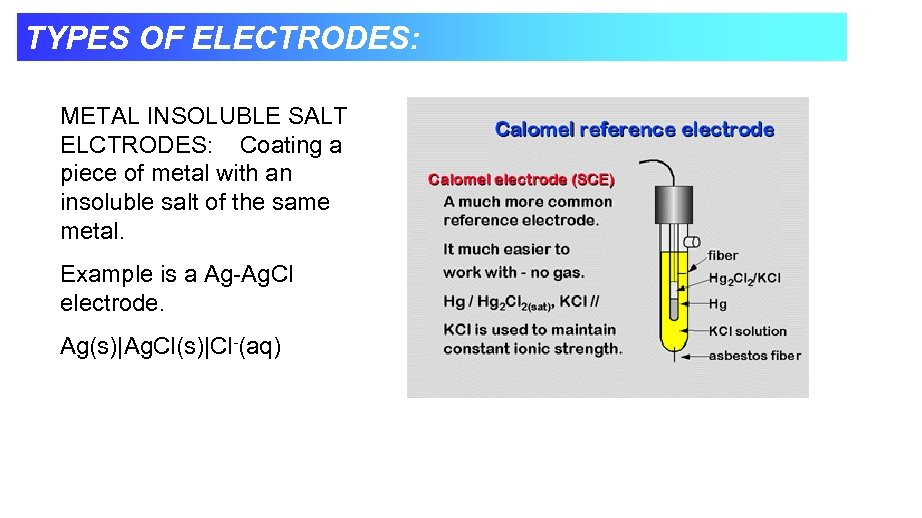TYPES OF ELECTRODES: METAL INSOLUBLE SALT ELCTRODES: Coating a piece of metal with an insoluble salt of the same metal. Example is a Ag-Ag. Cl electrode. Ag(s)|Ag. Cl(s)|Cl-(aq)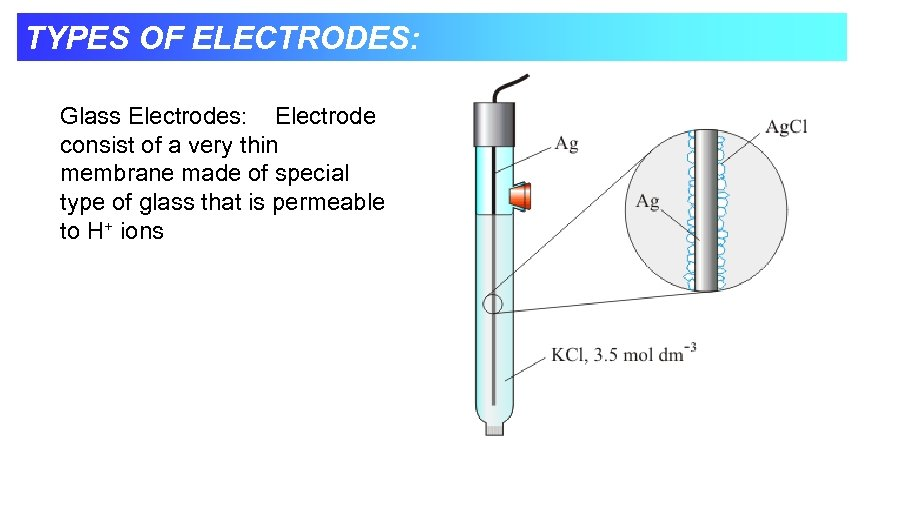TYPES OF ELECTRODES: Glass Electrodes: Electrode consist of a very thin membrane made of special type of glass that is permeable to H+ ions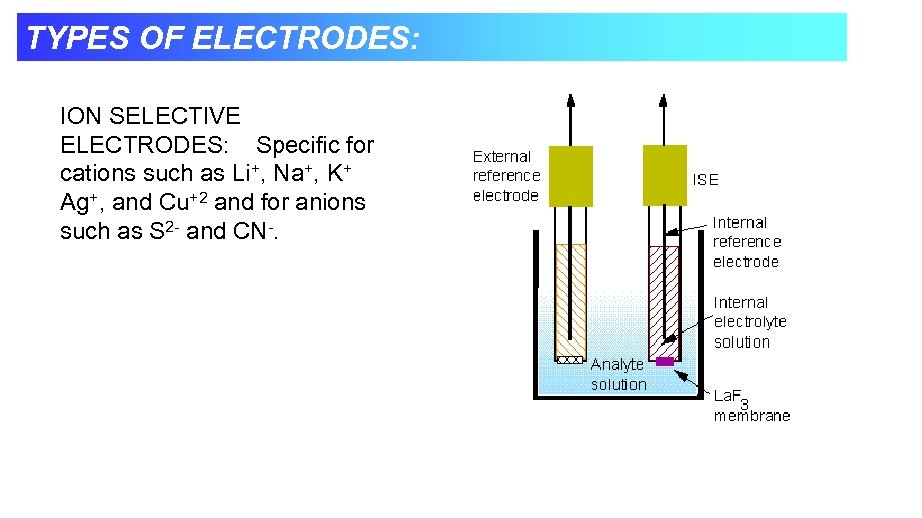TYPES OF ELECTRODES: ION SELECTIVE ELECTRODES: Specific for cations such as Li+, Na+, K+ Ag+, and Cu+2 and for anions such as S 2 - and CN-.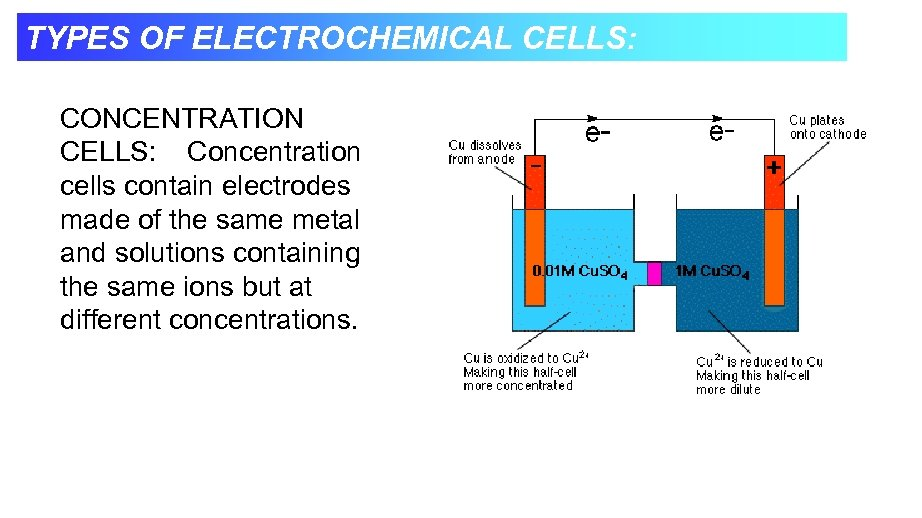TYPES OF ELECTROCHEMICAL CELLS: CONCENTRATION CELLS: Concentration cells contain electrodes made of the same metal and solutions containing the same ions but at different concentrations.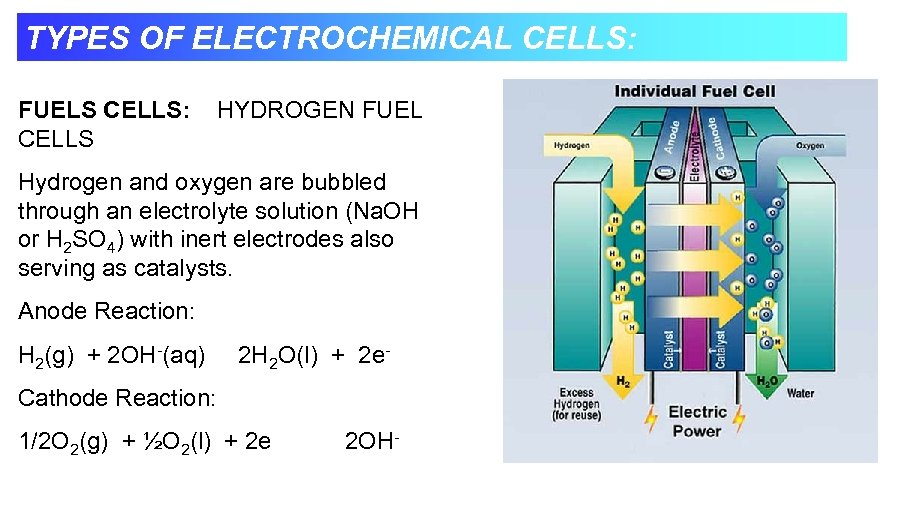TYPES OF ELECTROCHEMICAL CELLS: FUELS CELLS: CELLS HYDROGEN FUEL Hydrogen and oxygen are bubbled through an electrolyte solution (Na. OH or H 2 SO 4) with inert electrodes also serving as catalysts. Anode Reaction: H 2(g) + 2 OH-(aq) 2 H 2 O(l) + 2 e- Cathode Reaction: 1/2 O 2(g) + ½O 2(l) + 2 e 2 OH-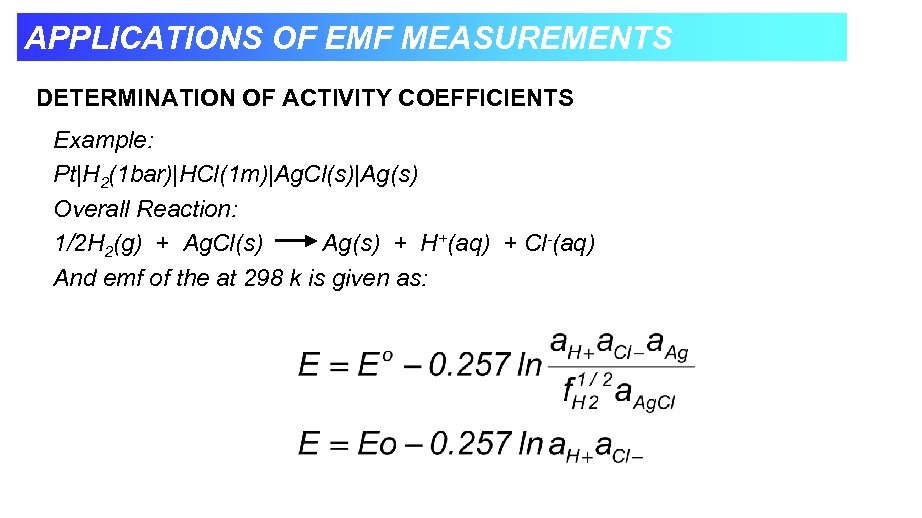APPLICATIONS OF EMF MEASUREMENTS DETERMINATION OF ACTIVITY COEFFICIENTS Example: Pt|H 2(1 bar)|HCl(1 m)|Ag. Cl(s)|Ag(s) Overall Reaction: 1/2 H 2(g) + Ag. Cl(s) Ag(s) + H+(aq) + Cl-(aq) And emf of the at 298 k is given as: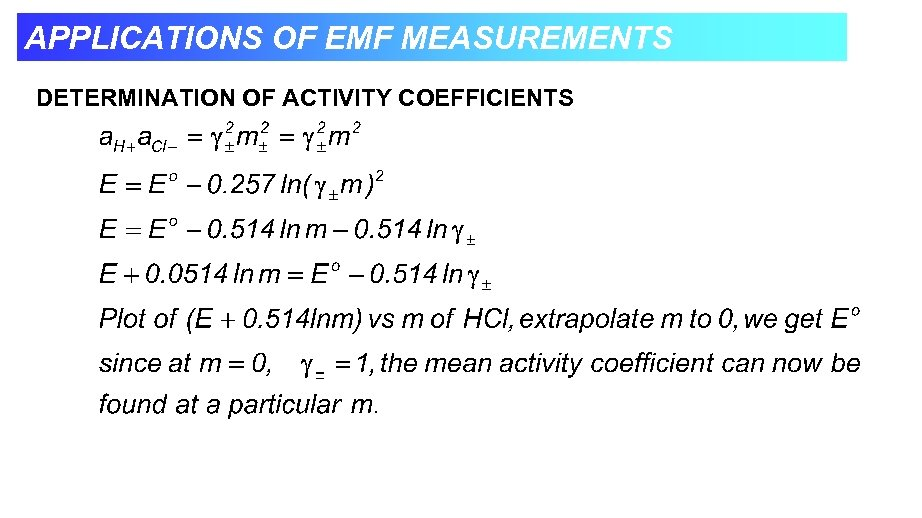APPLICATIONS OF EMF MEASUREMENTS DETERMINATION OF ACTIVITY COEFFICIENTS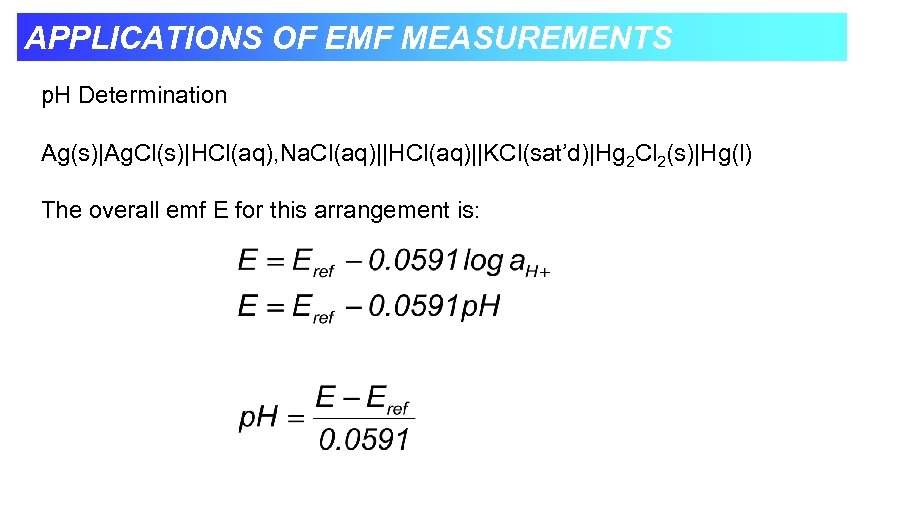APPLICATIONS OF EMF MEASUREMENTS p. H Determination Ag(s)|Ag. Cl(s)|HCl(aq), Na. Cl(aq)||HCl(aq)||KCl(sat’d)|Hg 2 Cl 2(s)|Hg(l) The overall emf E for this arrangement is: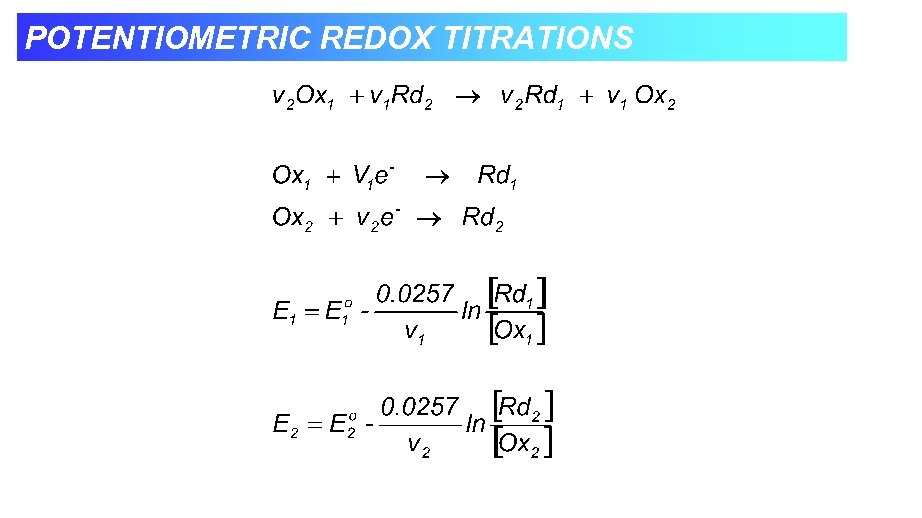POTENTIOMETRIC REDOX TITRATIONS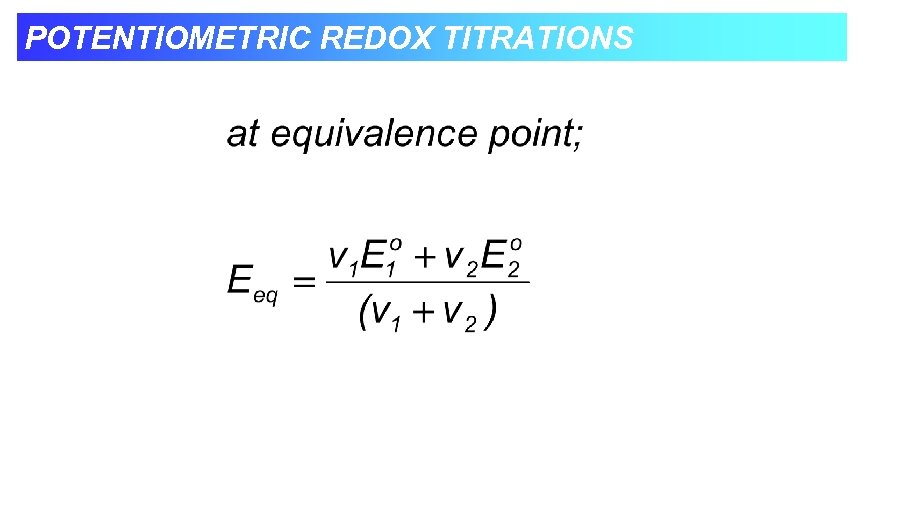POTENTIOMETRIC REDOX TITRATIONS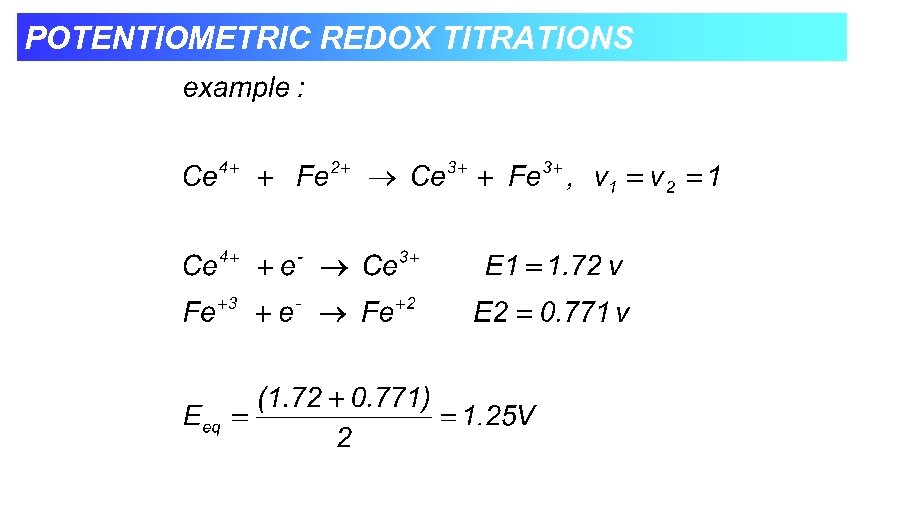POTENTIOMETRIC REDOX TITRATIONS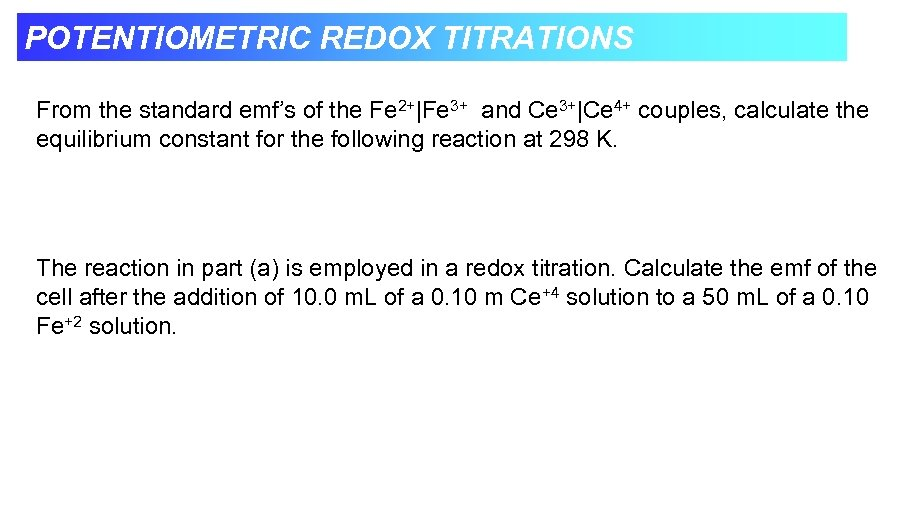POTENTIOMETRIC REDOX TITRATIONS From the standard emf’s of the Fe 2+|Fe 3+ and Ce 3+|Ce 4+ couples, calculate the equilibrium constant for the following reaction at 298 K. The reaction in part (a) is employed in a redox titration. Calculate the emf of the cell after the addition of 10. 0 m. L of a 0. 10 m Ce+4 solution to a 50 m. L of a 0. 10 Fe+2 solution.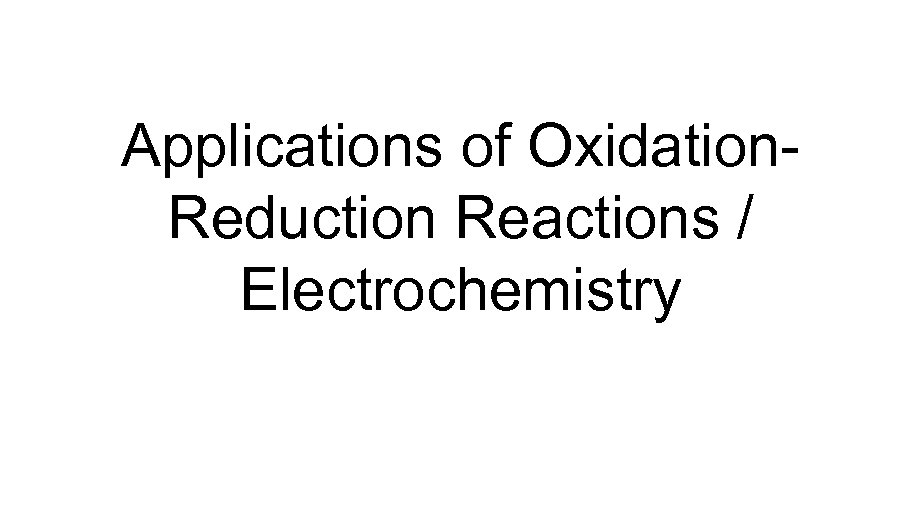Applications of Oxidation. Reduction Reactions / Electrochemistry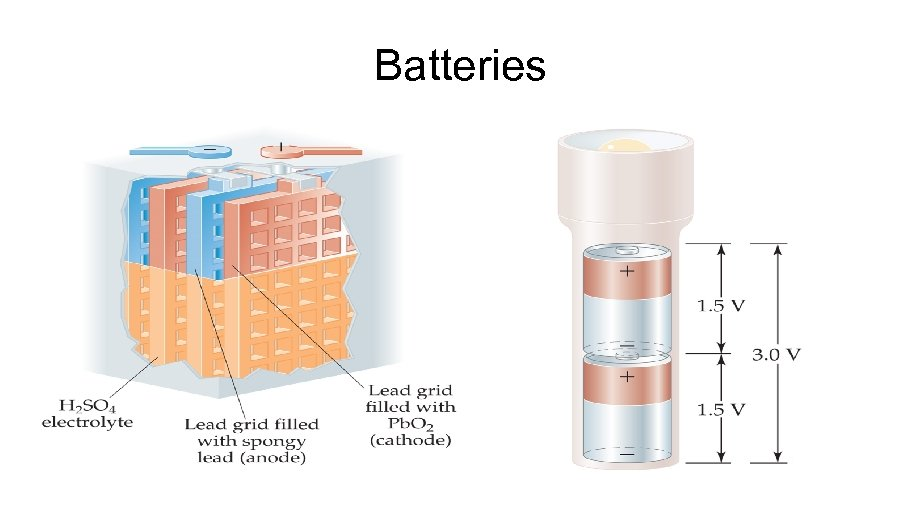Batteries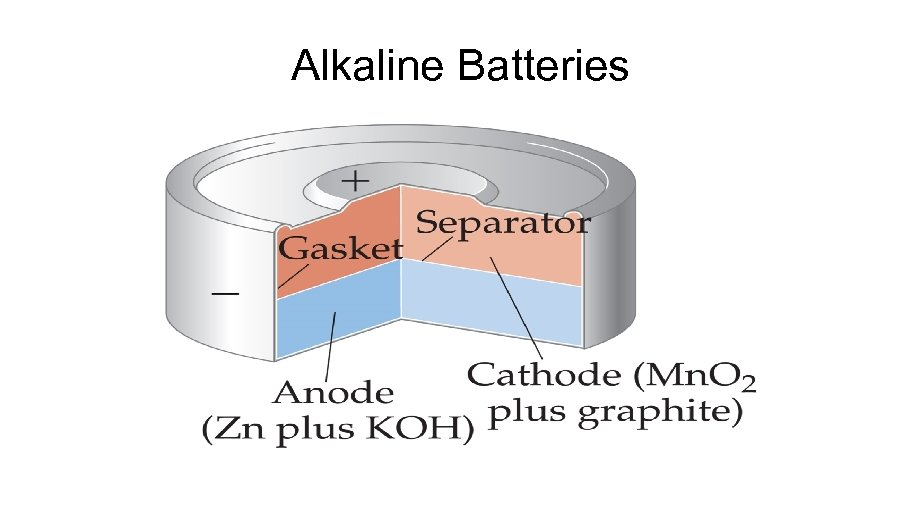Alkaline Batteries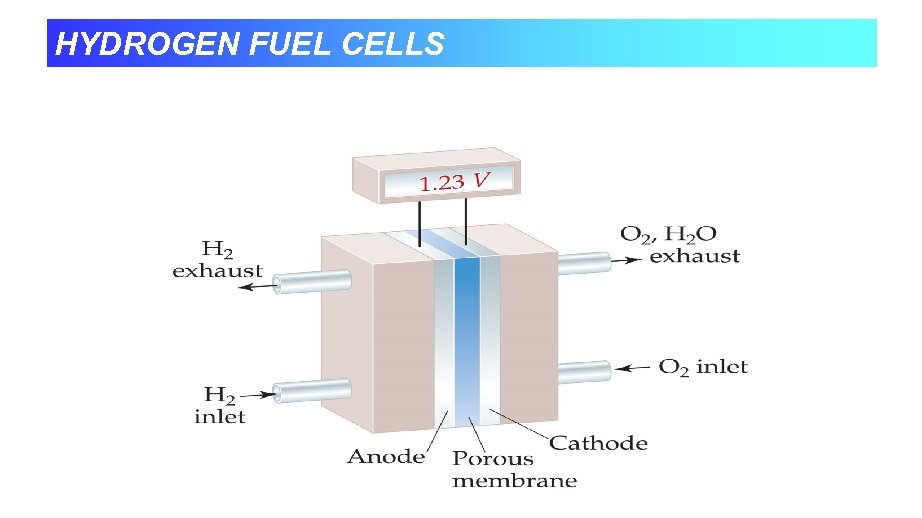HYDROGEN FUEL CELLS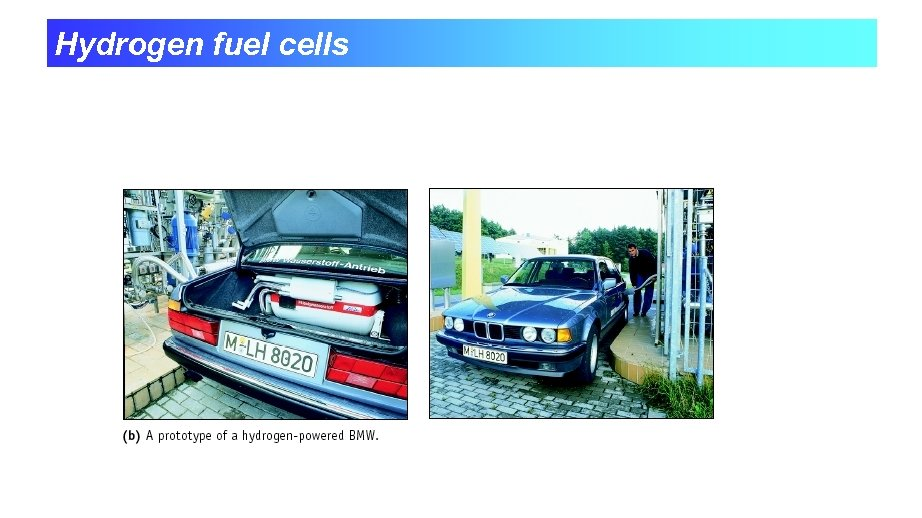Hydrogen fuel cells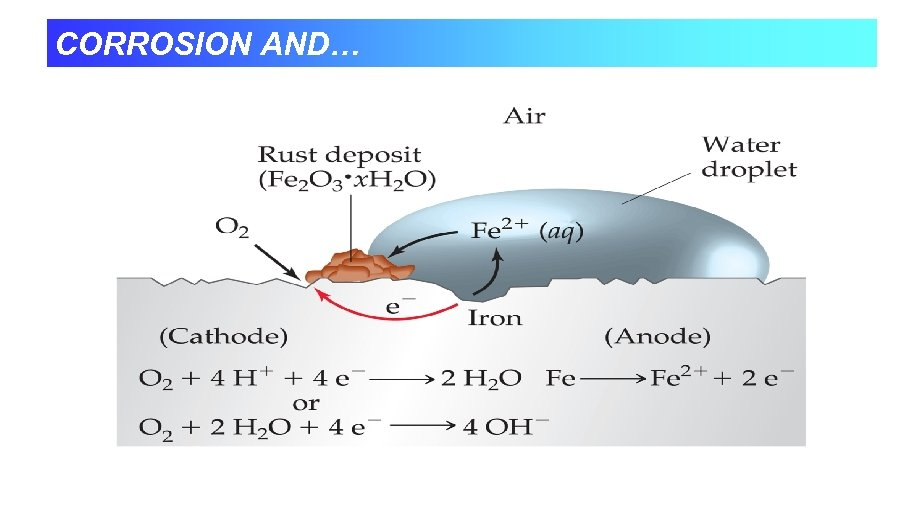CORROSION AND…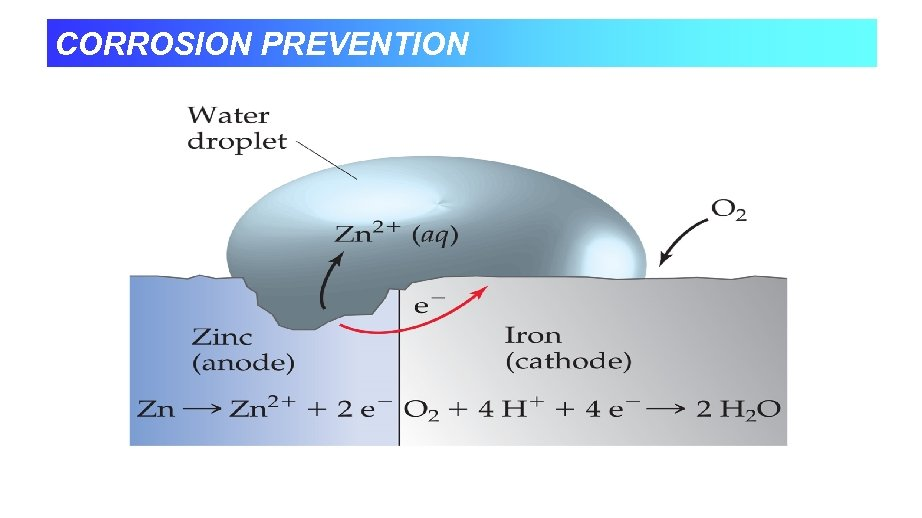CORROSION PREVENTION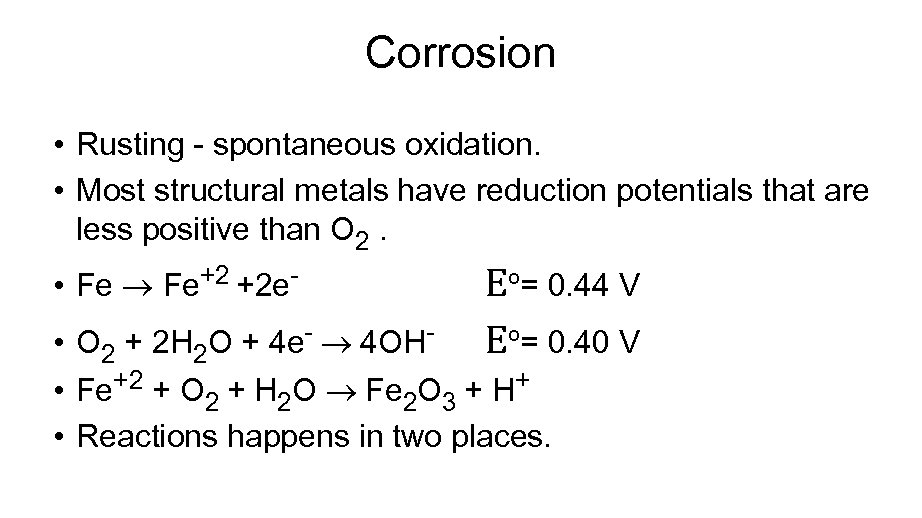Corrosion • Rusting - spontaneous oxidation. • Most structural metals have reduction potentials that are less positive than O 2. • Fe Fe+2 +2 e- Eº= 0. 44 V Eº= 0. 40 V • O 2 + 2 H 2 O + 4 e- 4 OH • Fe+2 + O 2 + H 2 O Fe 2 O 3 + H+ • Reactions happens in two places.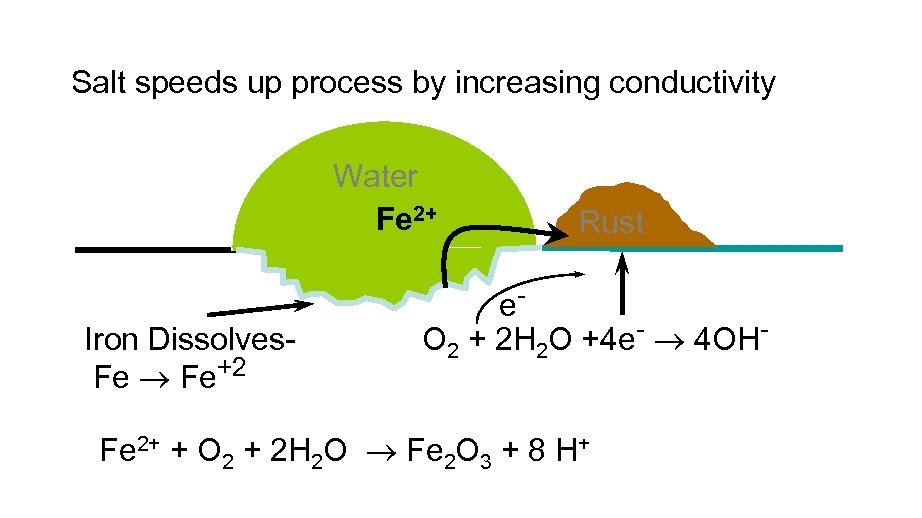Salt speeds up process by increasing conductivity Water Fe 2+ Iron Dissolves. Fe Fe+2 Rust e. O 2 + 2 H 2 O +4 e- 4 OH- Fe 2+ + O 2 + 2 H 2 O Fe 2 O 3 + 8 H+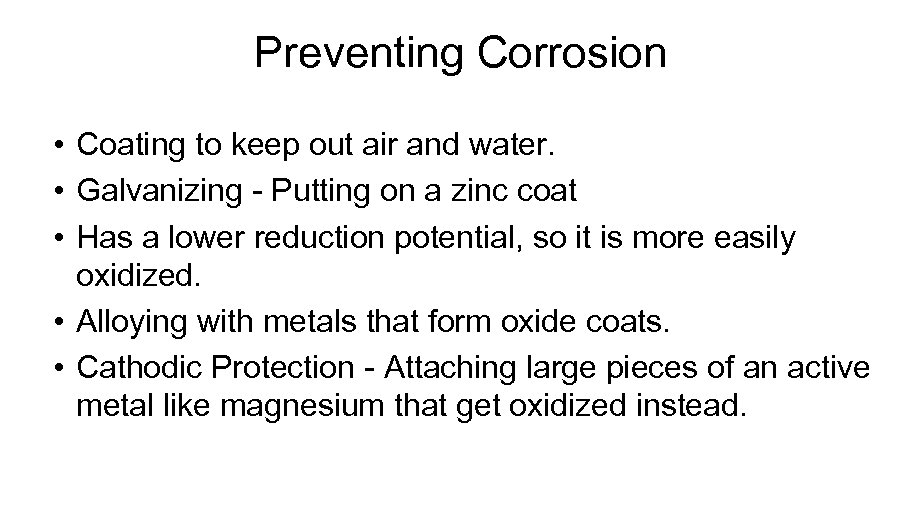Preventing Corrosion • Coating to keep out air and water. • Galvanizing - Putting on a zinc coat • Has a lower reduction potential, so it is more easily oxidized. • Alloying with metals that form oxide coats. • Cathodic Protection - Attaching large pieces of an active metal like magnesium that get oxidized instead.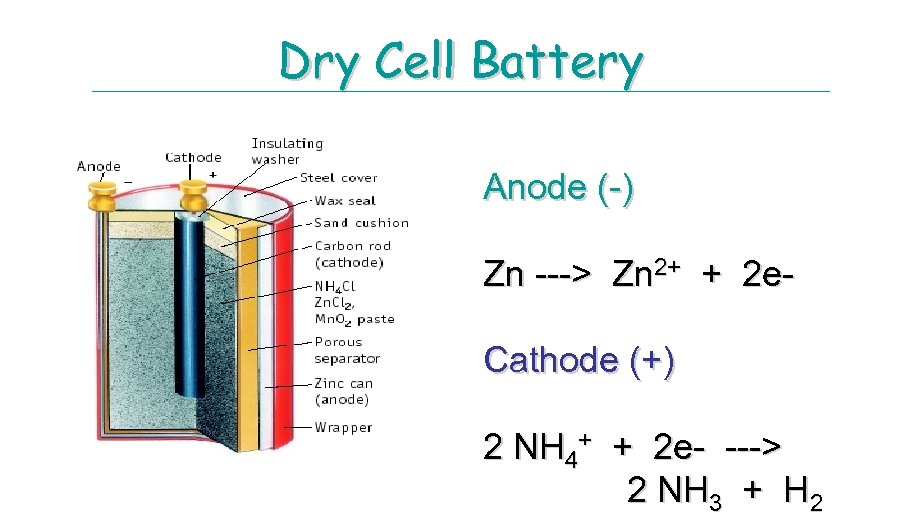Dry Cell Battery Anode (-) Zn ---> Zn 2+ + 2 e. Cathode (+) 2 NH 4+ + 2 e- ---> 2 NH 3 + H 2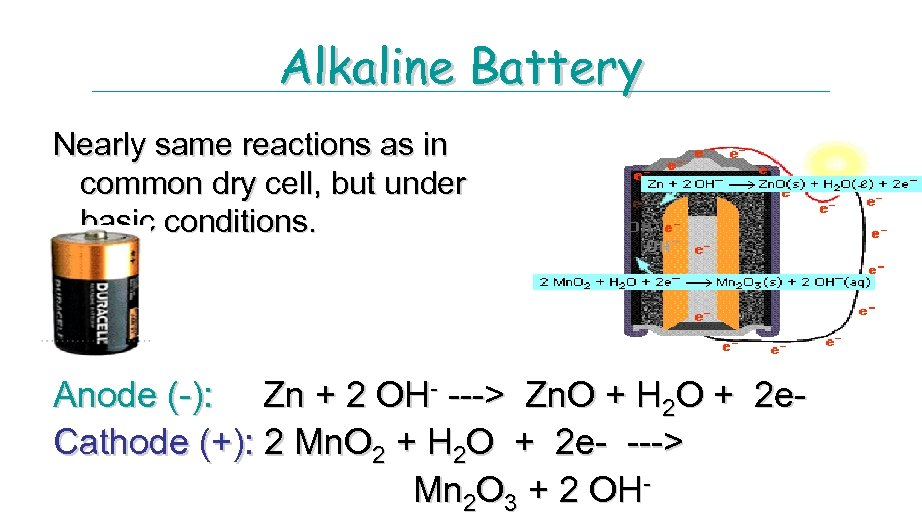Alkaline Battery Nearly same reactions as in common dry cell, but under basic conditions. Anode (-): Zn + 2 OH- ---> Zn. O + H 2 O + 2 e. Cathode (+): 2 Mn. O 2 + H 2 O + 2 e- ---> Mn 2 O 3 + 2 OH-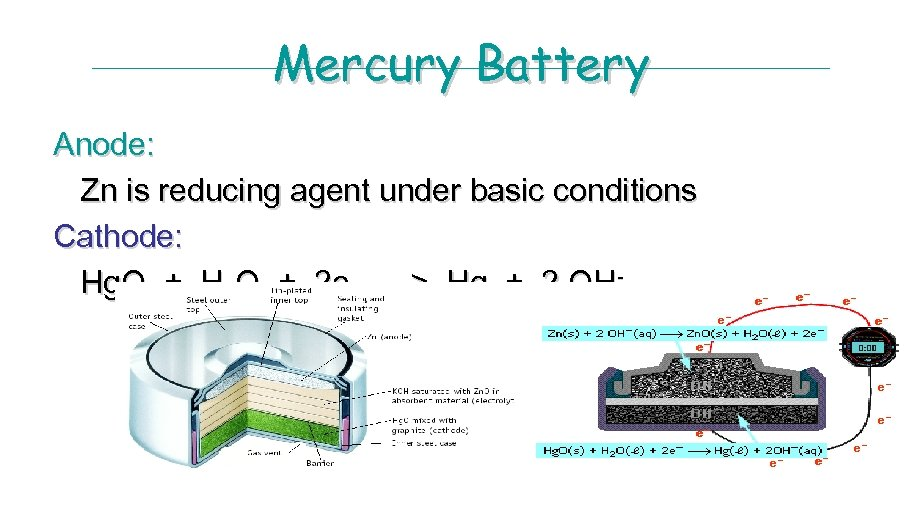Mercury Battery Anode: Zn is reducing agent under basic conditions Cathode: Hg. O + H 2 O + 2 e- ---> Hg + 2 OH-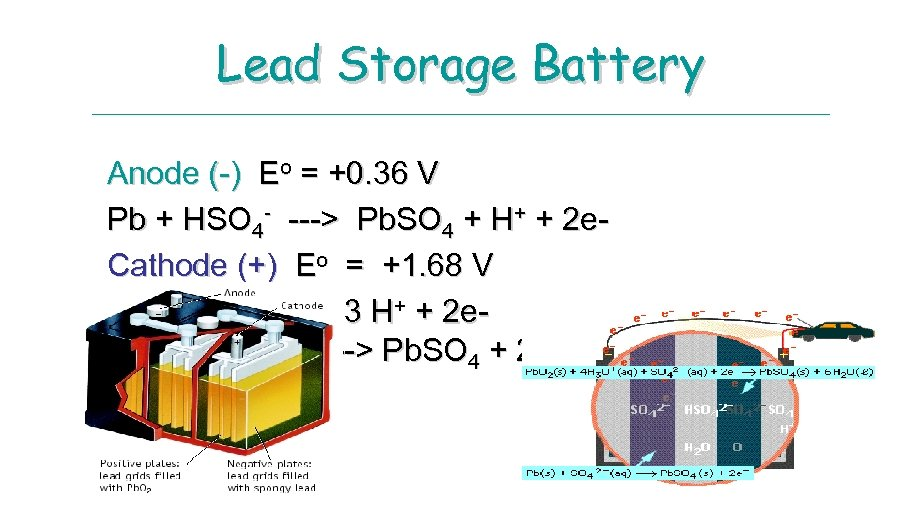Lead Storage Battery Anode (-) Eo = +0. 36 V Pb + HSO 4 - ---> Pb. SO 4 + H+ + 2 e. Cathode (+) Eo = +1. 68 V Pb. O 2 + HSO 4 - + 3 H+ + 2 e---> Pb. SO 4 + 2 H 2 O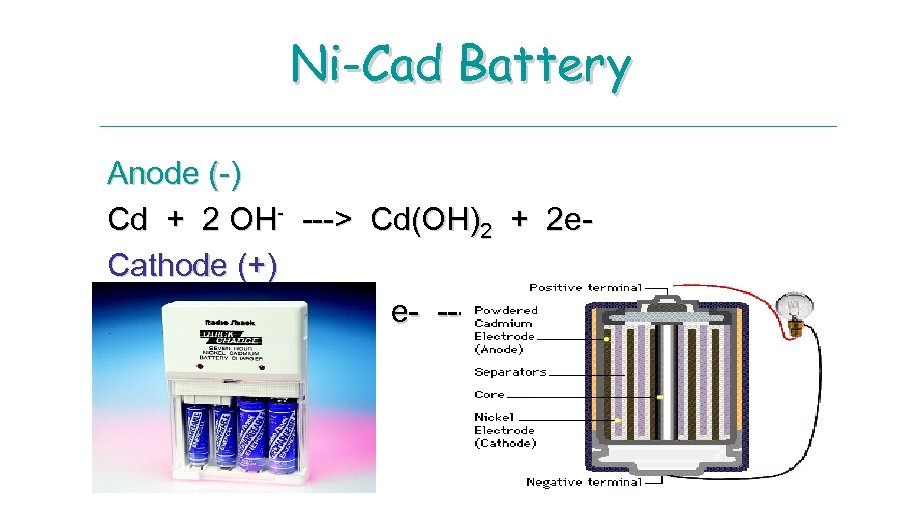Ni-Cad Battery Anode (-) Cd + 2 OH- ---> Cd(OH)2 + 2 e. Cathode (+) Ni. O(OH) + H 2 O + e- ---> Ni(OH)2 + OH-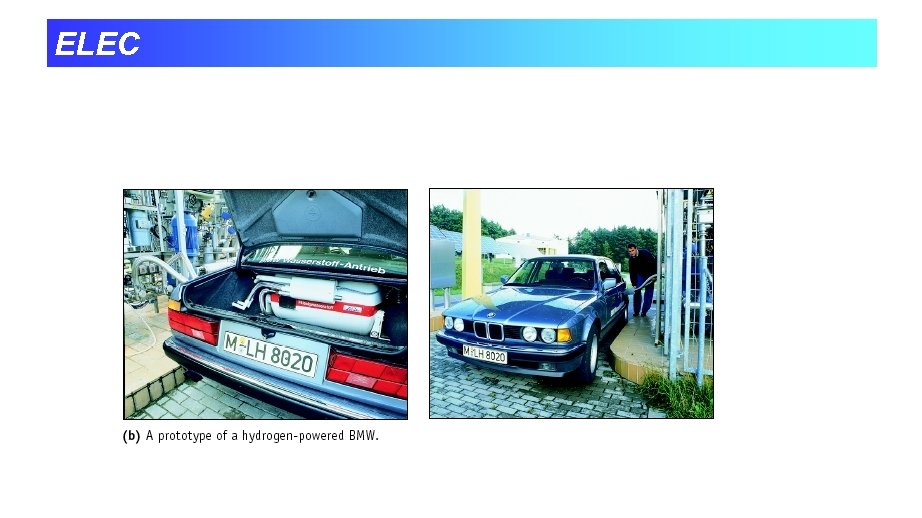ELEC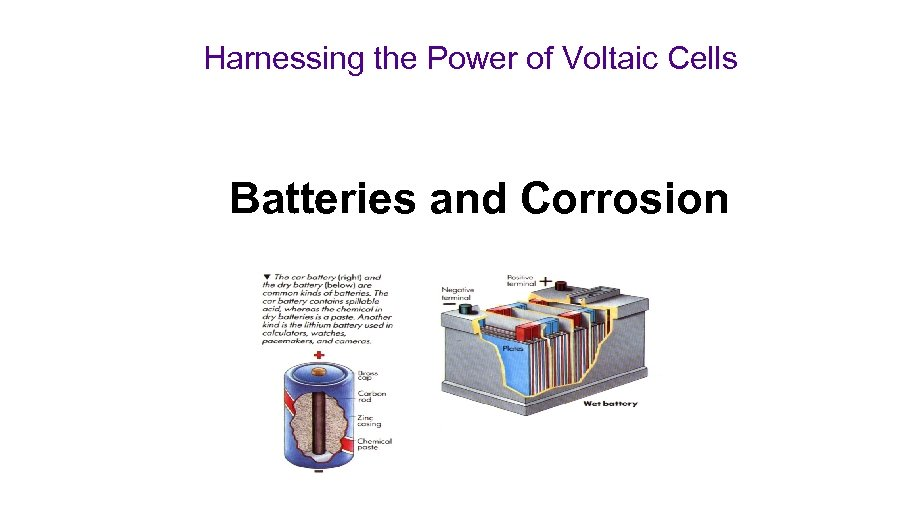Harnessing the Power of Voltaic Cells Batteries and Corrosion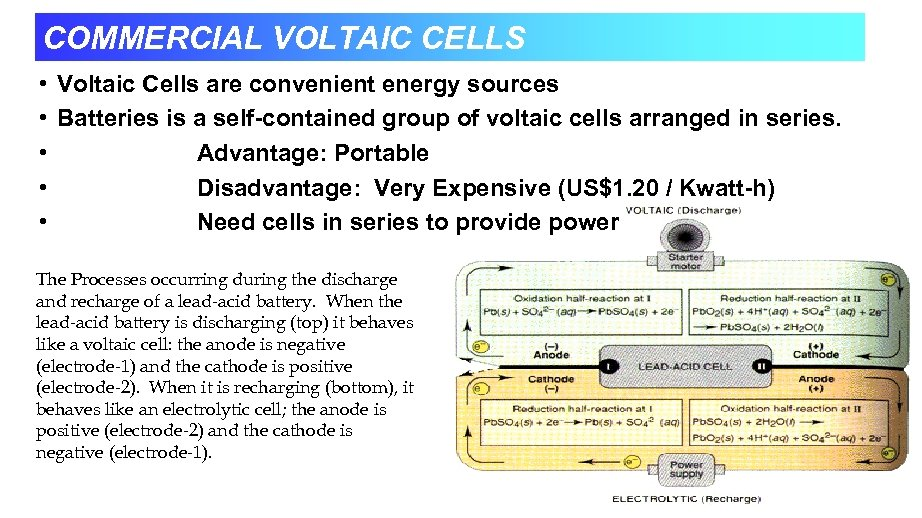COMMERCIAL VOLTAIC CELLS • Voltaic Cells are convenient energy sources • Batteries is a self-contained group of voltaic cells arranged in series. • Advantage: Portable • Disadvantage: Very Expensive (US\$1. 20 / Kwatt-h) • Need cells in series to provide power The Processes occurring during the discharge and recharge of a lead-acid battery. When the lead-acid battery is discharging (top) it behaves like a voltaic cell: the anode is negative (electrode-1) and the cathode is positive (electrode-2). When it is recharging (bottom), it behaves like an electrolytic cell; the anode is positive (electrode-2) and the cathode is negative (electrode-1).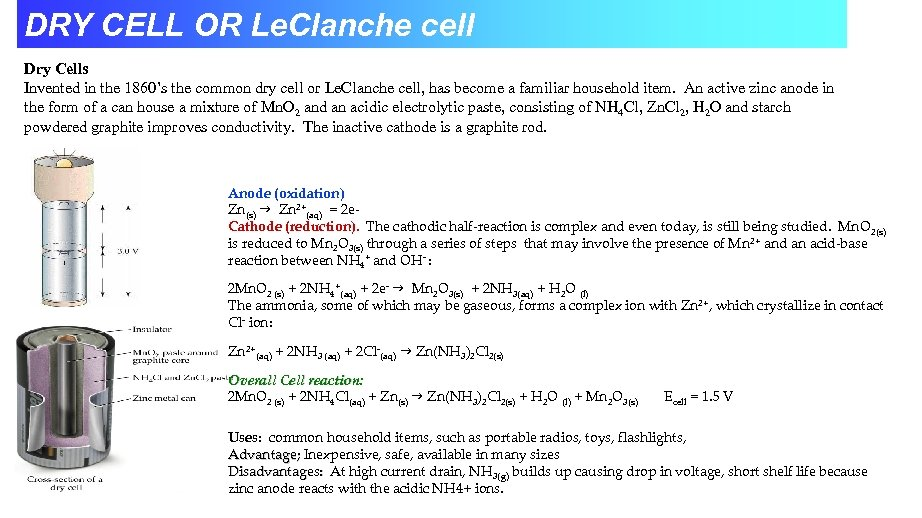DRY CELL OR Le. Clanche cell Dry Cells Invented in the 1860’s the common dry cell or Le. Clanche cell, has become a familiar household item. An active zinc anode in the form of a can house a mixture of Mn. O 2 and an acidic electrolytic paste, consisting of NH 4 Cl, Zn. Cl 2, H 2 O and starch powdered graphite improves conductivity. The inactive cathode is a graphite rod. Anode (oxidation) Zn(s) g Zn 2+(aq) = 2 e. Cathode (reduction). The cathodic half-reaction is complex and even today, is still being studied. Mn. O 2(s) is reduced to Mn 2 O 3(s) through a series of steps that may involve the presence of Mn 2+ and an acid-base reaction between NH 4+ and OH- : 2 Mn. O 2 (s) + 2 NH 4+(aq) + 2 e- g Mn 2 O 3(s) + 2 NH 3(aq) + H 2 O (l) The ammonia, some of which may be gaseous, forms a complex ion with Zn 2+, which crystallize in contact Cl- ion: Zn 2+(aq) + 2 NH 3 (aq) + 2 Cl-(aq) g Zn(NH 3)2 Cl 2(s) Overall Cell reaction: 2 Mn. O 2 (s) + 2 NH 4 Cl(aq) + Zn(s) g Zn(NH 3)2 Cl 2(s) + H 2 O (l) + Mn 2 O 3(s) Ecell = 1. 5 V Uses: common household items, such as portable radios, toys, flashlights, Advantage; Inexpensive, safe, available in many sizes Disadvantages: At high current drain, NH 3(g) builds up causing drop in voltage, short shelf life because zinc anode reacts with the acidic NH 4+ ions.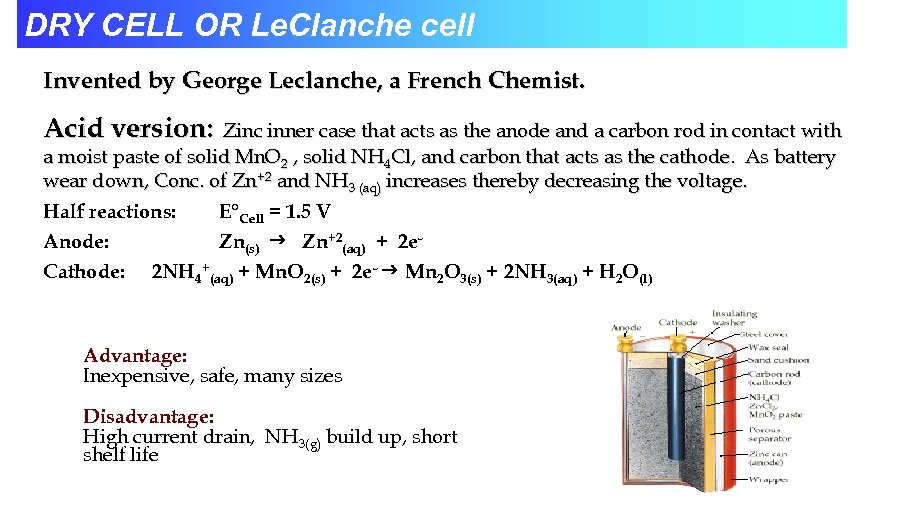DRY CELL OR Le. Clanche cell Invented by George Leclanche, a French Chemist. Acid version: Zinc inner case that acts as the anode and a carbon rod in contact with a moist paste of solid Mn. O 2 , solid NH 4 Cl, and carbon that acts as the cathode. As battery wear down, Conc. of Zn+2 and NH 3 (aq) increases thereby decreasing the voltage. Half reactions: E°Cell = 1. 5 V Anode: Zn(s) Zn+2(aq) + 2 e. Cathode: 2 NH 4+(aq) + Mn. O 2(s) + 2 e- Mn 2 O 3(s) + 2 NH 3(aq) + H 2 O(l) Advantage: Inexpensive, safe, many sizes Disadvantage: High current drain, NH 3(g) build up, short shelf life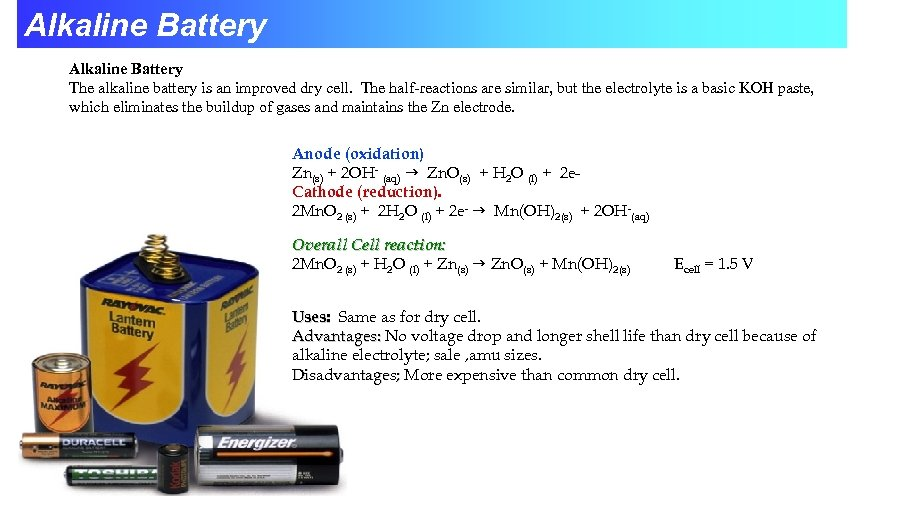Alkaline Battery The alkaline battery is an improved dry cell. The half-reactions are similar, but the electrolyte is a basic KOH paste, which eliminates the buildup of gases and maintains the Zn electrode. Anode (oxidation) Zn(s) + 2 OH- (aq) g Zn. O(s) + H 2 O (l) + 2 e. Cathode (reduction). 2 Mn. O 2 (s) + 2 H 2 O (l) + 2 e- g Mn(OH)2(s) + 2 OH-(aq) Overall Cell reaction: 2 Mn. O 2 (s) + H 2 O (l) + Zn(s) g Zn. O(s) + Mn(OH)2(s) Ecell = 1. 5 V Uses: Same as for dry cell. Advantages: No voltage drop and longer shell life than dry cell because of alkaline electrolyte; sale , amu sizes. Disadvantages; More expensive than common dry cell.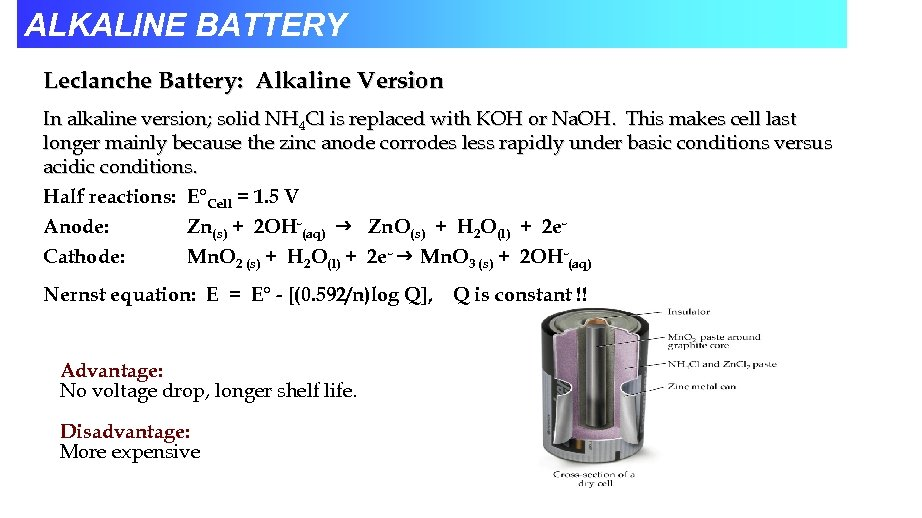ALKALINE BATTERY Leclanche Battery: Alkaline Version In alkaline version; solid NH 4 Cl is replaced with KOH or Na. OH. This makes cell last longer mainly because the zinc anode corrodes less rapidly under basic conditions versus acidic conditions. Half reactions: E°Cell = 1. 5 V Anode: Zn(s) + 2 OH-(aq) Zn. O(s) + H 2 O(l) + 2 e. Cathode: Mn. O 2 (s) + H 2 O(l) + 2 e- Mn. O 3 (s) + 2 OH-(aq) Nernst equation: E = E° - [(0. 592/n)log Q], Advantage: No voltage drop, longer shelf life. Disadvantage: More expensive Q is constant !!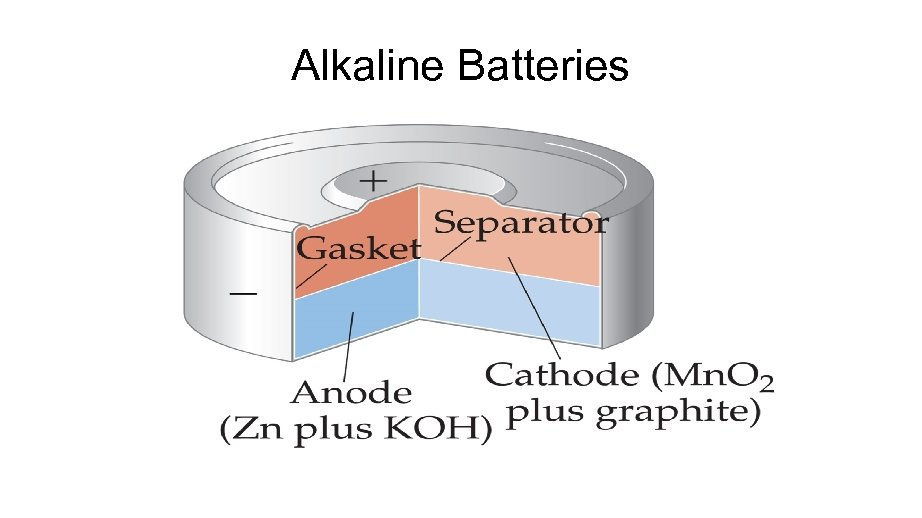Alkaline Batteries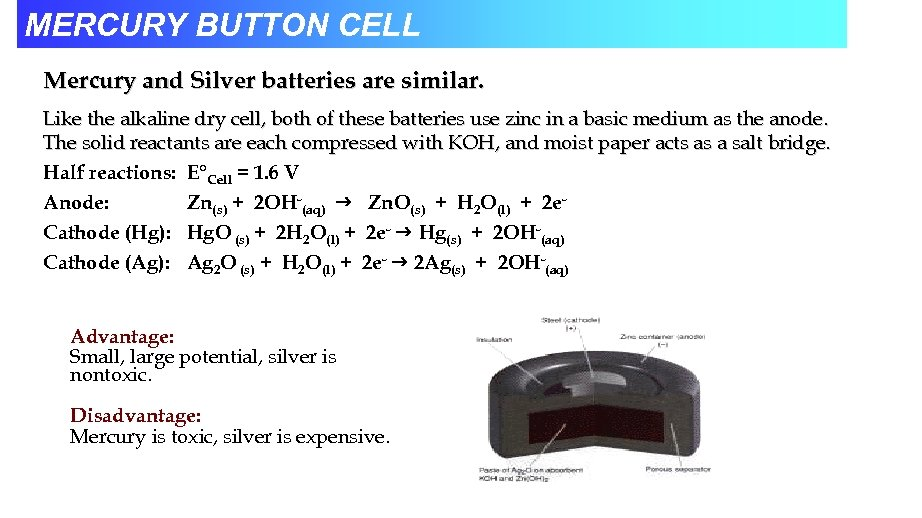MERCURY BUTTON CELL Mercury and Silver batteries are similar. Like the alkaline dry cell, both of these batteries use zinc in a basic medium as the anode. The solid reactants are each compressed with KOH, and moist paper acts as a salt bridge. Half reactions: E°Cell = 1. 6 V Anode: Zn(s) + 2 OH-(aq) Zn. O(s) + H 2 O(l) + 2 e. Cathode (Hg): Hg. O (s) + 2 H 2 O(l) + 2 e- Hg(s) + 2 OH-(aq) Cathode (Ag): Ag 2 O (s) + H 2 O(l) + 2 e- 2 Ag(s) + 2 OH-(aq) Advantage: Small, large potential, silver is nontoxic. Disadvantage: Mercury is toxic, silver is expensive.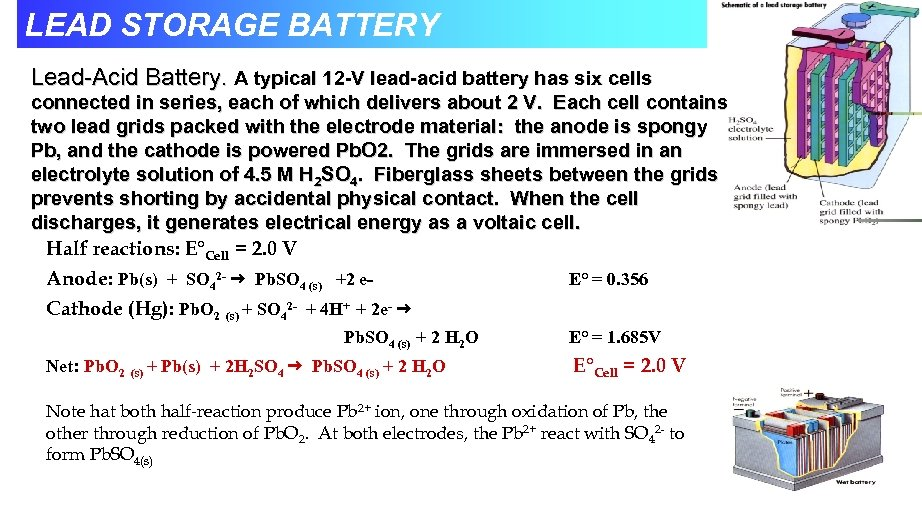LEAD STORAGE BATTERY Lead-Acid Battery. A typical 12 -V lead-acid battery has six cells connected in series, each of which delivers about 2 V. Each cell contains two lead grids packed with the electrode material: the anode is spongy Pb, and the cathode is powered Pb. O 2. The grids are immersed in an electrolyte solution of 4. 5 M H 2 SO 4. Fiberglass sheets between the grids prevents shorting by accidental physical contact. When the cell discharges, it generates electrical energy as a voltaic cell. Half reactions: E°Cell = 2. 0 V Anode: Pb(s) + SO 42 - Pb. SO 4 (s) +2 e. E° = 0. 356 Cathode (Hg): Pb. O 2 (s) + SO 42 - + 4 H+ + 2 e- Pb. SO 4 (s) + 2 H 2 O Net: Pb. O 2 (s) + Pb(s) + 2 H 2 SO 4 Pb. SO 4 (s) + 2 H 2 O E° = 1. 685 V E°Cell = 2. 0 V Note hat both half-reaction produce Pb 2+ ion, one through oxidation of Pb, the other through reduction of Pb. O 2. At both electrodes, the Pb 2+ react with SO 42 - to form Pb. SO 4(s)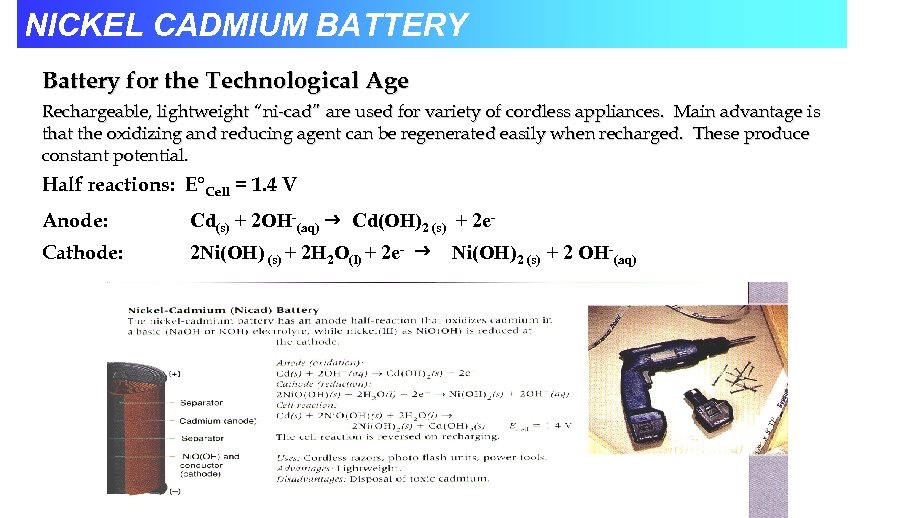NICKEL CADMIUM BATTERY Battery for the Technological Age Rechargeable, lightweight “ni-cad” are used for variety of cordless appliances. Main advantage is that the oxidizing and reducing agent can be regenerated easily when recharged. These produce constant potential. Half reactions: E°Cell = 1. 4 V Anode: Cd(s) + 2 OH-(aq) Cd(OH)2 (s) + 2 e- Cathode: 2 Ni(OH) (s) + 2 H 2 O(l) + 2 e- Ni(OH)2 (s) + 2 OH-(aq)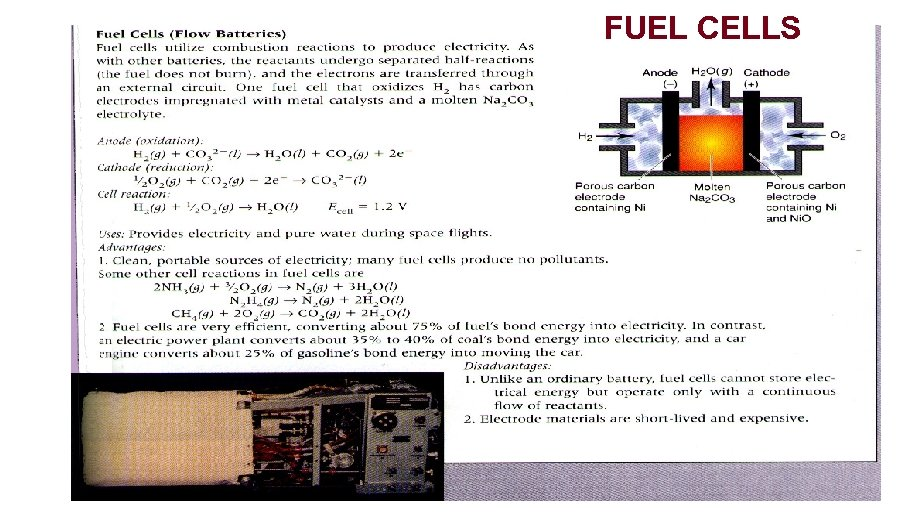FUEL CELLS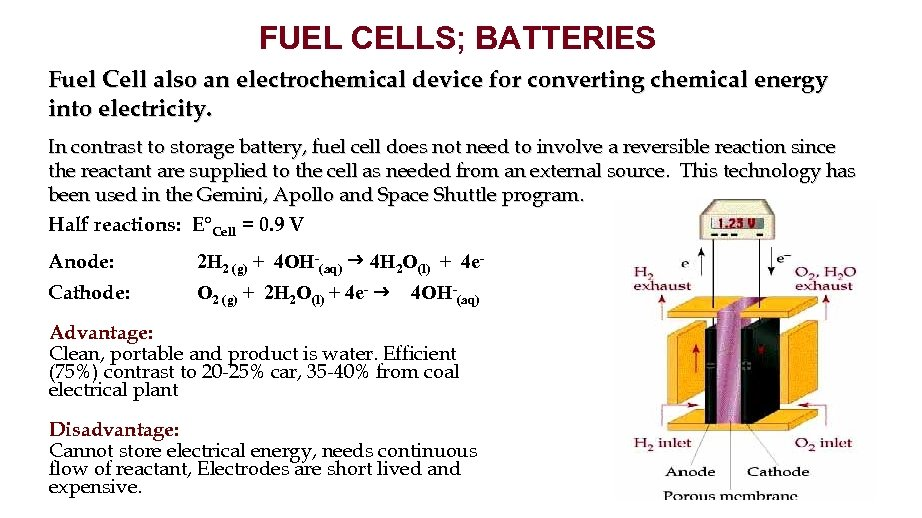FUEL CELLS; BATTERIES Fuel Cell also an electrochemical device for converting chemical energy into electricity. In contrast to storage battery, fuel cell does not need to involve a reversible reaction since the reactant are supplied to the cell as needed from an external source. This technology has been used in the Gemini, Apollo and Space Shuttle program. Half reactions: E°Cell = 0. 9 V Anode: 2 H 2 (g) + 4 OH-(aq) 4 H 2 O(l) + 4 e- Cathode: O 2 (g) + 2 H 2 O(l) + 4 e- 4 OH-(aq) Advantage: Clean, portable and product is water. Efficient (75%) contrast to 20 -25% car, 35 -40% from coal electrical plant Disadvantage: Cannot store electrical energy, needs continuous flow of reactant, Electrodes are short lived and expensive.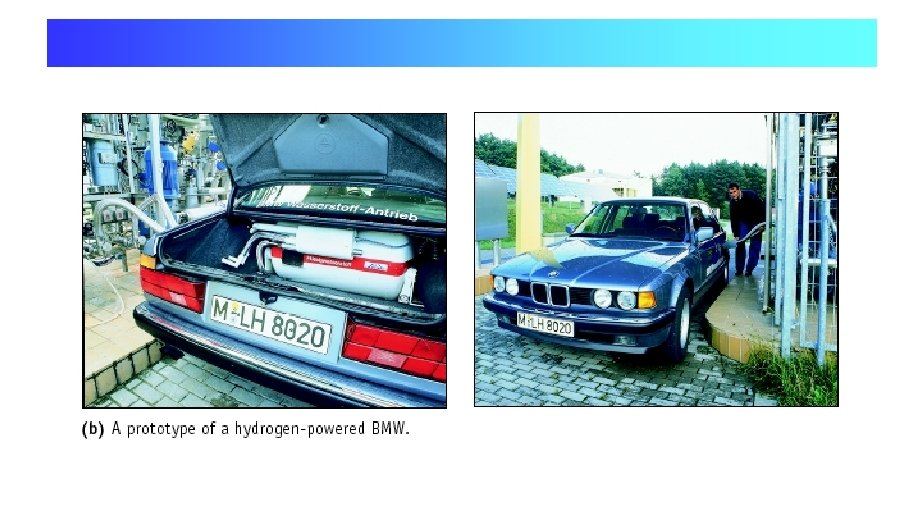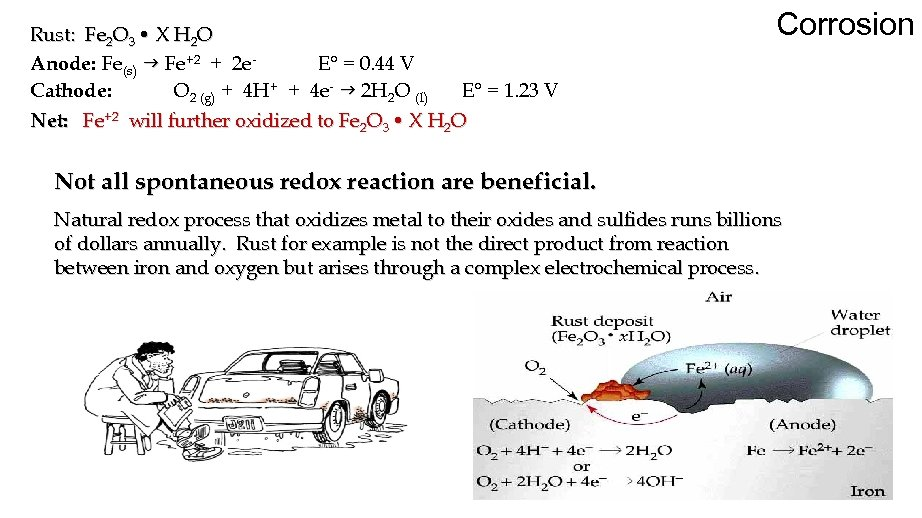Rust: Fe 2 O 3 • X H 2 O Anode: Fe(s) g Fe+2 + 2 e. E° = 0. 44 V Cathode: O 2 (g) + 4 H+ + 4 e- g 2 H 2 O (l) E° = 1. 23 V Net: Fe+2 will further oxidized to Fe 2 O 3 • X H 2 O Corrosion Not all spontaneous redox reaction are beneficial. Natural redox process that oxidizes metal to their oxides and sulfides runs billions of dollars annually. Rust for example is not the direct product from reaction between iron and oxygen but arises through a complex electrochemical process.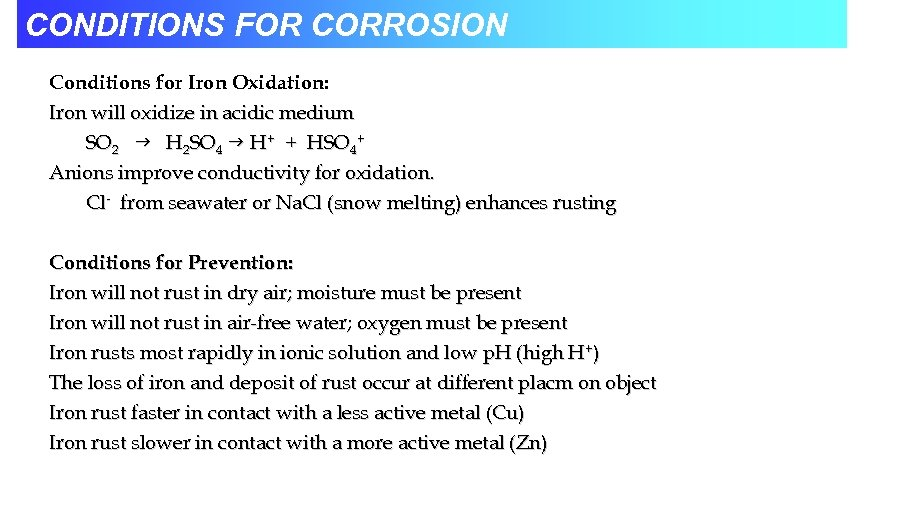CONDITIONS FOR CORROSION Conditions for Iron Oxidation: Iron will oxidize in acidic medium SO 2 g H 2 SO 4 g H+ + HSO 4+ Anions improve conductivity for oxidation. Cl- from seawater or Na. Cl (snow melting) enhances rusting Conditions for Prevention: Iron will not rust in dry air; moisture must be present Iron will not rust in air-free water; oxygen must be present Iron rusts most rapidly in ionic solution and low p. H (high H+) The loss of iron and deposit of rust occur at different placm on object Iron rust faster in contact with a less active metal (Cu) Iron rust slower in contact with a more active metal (Zn)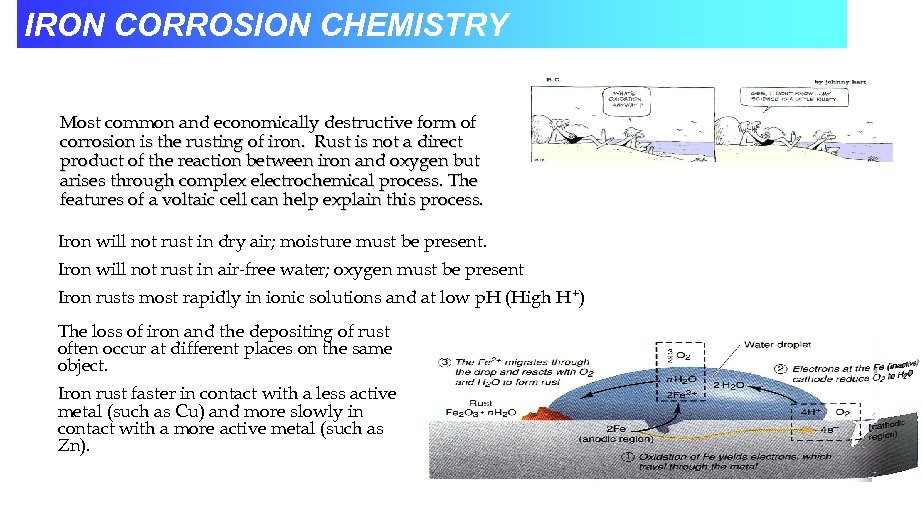IRON CORROSION CHEMISTRY Most common and economically destructive form of corrosion is the rusting of iron. Rust is not a direct product of the reaction between iron and oxygen but arises through complex electrochemical process. The features of a voltaic cell can help explain this process. Iron will not rust in dry air; moisture must be present. Iron will not rust in air-free water; oxygen must be present Iron rusts most rapidly in ionic solutions and at low p. H (High H+) The loss of iron and the depositing of rust often occur at different places on the same object. Iron rust faster in contact with a less active metal (such as Cu) and more slowly in contact with a more active metal (such as Zn).CORROSION AND…CORROSION PREVENTION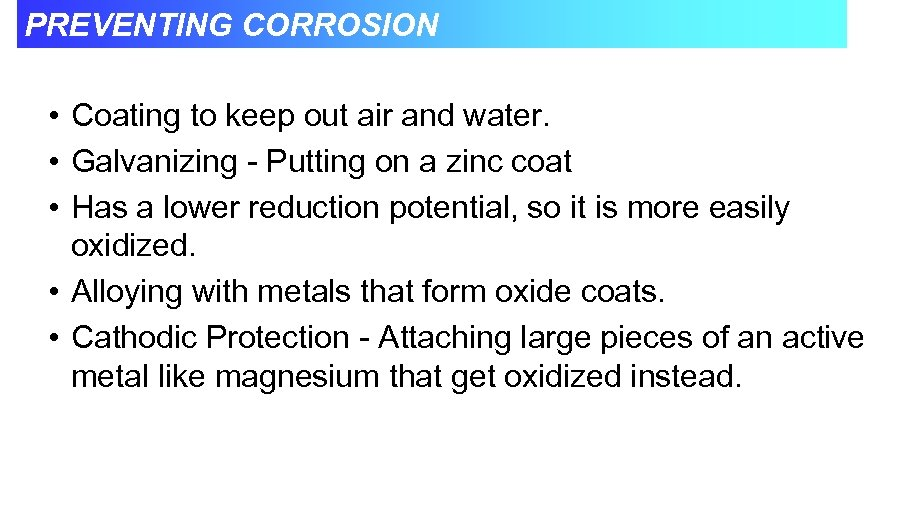PREVENTING CORROSION • Coating to keep out air and water. • Galvanizing - Putting on a zinc coat • Has a lower reduction potential, so it is more easily oxidized. • Alloying with metals that form oxide coats. • Cathodic Protection - Attaching large pieces of an active metal like magnesium that get oxidized instead.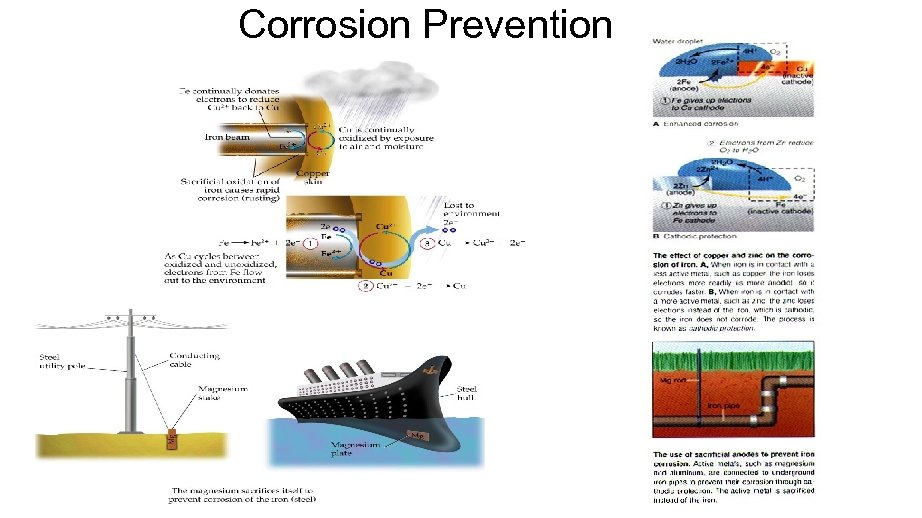Corrosion Prevention